# Strings, Arrays & Linked Lists

Array-based problems are the hardest problems by far there are way too many ways of manipulating an array or traversing it and so you have many different ways of actually approaching the problem in a sense you kind of get overloaded by all the possibilities.

## Common ways to solve problems

• Think of sorting the input array
• Think of two pointers

Notes on this:

Pointers

• Fast and slow
• Same speed
• Separate ends
• Both on one end
• Sliding window

Examples:

Examples:

# Strings

Python Strings

Strings

How to solve DP - String? Template and 4 Steps to be followed. - LeetCode Discuss

### Examples

• Valid Anagram

"""
Valid Anagram

Given two strings s and t, return true if t is an anagram of s, and false otherwise.

Example 1:

Input: s = "anagram", t = "nagaram"
Output: true
Example 2:

Input: s = "rat", t = "car"
Output: false

https://leetcode.com/problems/valid-anagram/
"""

# 0(n) time | O(n) space
class Solution:
def isAnagram(self, s: str, t: str):

len_s = len(s)
len_t = len(t)

if len_s != len_t:
return False

# chars from s will be added as +1
# chars from t will be added as -1
# then we check if each char will have a total of 0
store = {}

for i in range(len_s):

# s
if s[i] not in store:
store[s[i]] = 1
else:
store[s[i]] = store[s[i]] + 1

# t
if t[i] not in store:
store[t[i]] = -1
else:
store[t[i]] = store[t[i]] - 1

# check if each character in the store has a value of 0
for char in s:
if store[char] != 0:
return False

return True

"""
Example 1:
Input: s = "anagram", t = "nagaram"
Output: true

Example 2:
Input: s = "rat", t = "car"
Output: false
"""

• Group Anagrams

"""
Group Anagrams:

Write a function that takes in an array of strings and groups anagrams together.
Anagrams are strings made up of exactly the same letters, where order doesn't matter.
For example, "cinema" and "iceman" are anagrams; similarly, "foo" and "ofo" are anagrams.
Your function should return a list of anagram groups in no particular order.

Sample Input
words = ["yo", "act", "flop", "tac", "foo", "cat", "oy", "olfp"]
Sample Output
[["yo", "oy"], ["flop", "olfp"], ["act", "tac", "cat"], ["foo"]]

https://www.algoexpert.io/questions/Group%20Anagrams
https://leetcode.com/problems/group-anagrams/
"""

"""
solution for groupAnagrams1:
1. create new array sorted_words with each string sorted in alphabetical order
2. create new array sorted_idxs with sorted_words indexes
3. sort sorted_idxs by the alphabetical order of the words in sorted_words
4. you have the sorted_words in alphabetical order...
- iterate though the sorted indeces grouping words that match together

words =        ['yo', 'act', 'flop', 'tac', 'foo', 'cat', 'oy', 'olfp']
sorted_words = ['oy', 'act', 'flop', 'act', 'foo', 'act', 'oy', 'flop']
sorted_idxs =  [0, 1, 2, 3, 4, 5, 6, 7]
sorted_idxs =  [1, 3, 5, 2, 7, 4, 0, 6]
"""

# O(w * n * log(n) + n * w * log(w)) time | O(wn) space
#   - where w is the number of words and n is the length of the longest word
def groupAnagrams1(words):

sorted_words = []
for word in words:
sorted_words.append("".join(sorted(word)))

sorted_idxs = list(range(len(words)))

sorted_idxs.sort(key=lambda idx: sorted_words[idx])

anagrams = []
idx = 0
while idx < len(sorted_idxs):
group = [words[sorted_idxs[idx]]]
idx += 1

while idx < len(sorted_idxs) and sorted_words[sorted_idxs[idx]] == sorted_words[sorted_idxs[idx-1]]:

group.append(words[sorted_idxs[idx]])
idx += 1

anagrams.append(group)

return anagrams

"""
solution for groupAnagrams2 & groupAnagrams:
1. create new array sorted_words with each string sorted in alphabetical order

create a store hashmap b4 2
2. iterate through sorted_words checking if we have seen it before
if not:
add it to the store atoring its index in an array which will be its value in the hasmap
else:
add it's index to the array that is it's value in the store
3. for each key in the store genarate its respective strings from the keys in the array

words =        ['yo', 'act', 'flop', 'tac', 'foo', 'cat', 'oy', 'olfp']
sorted_words = ['oy', 'act', 'flop', 'act', 'foo', 'act', 'oy', 'flop']

"""

def groupAnagrams2(words):

sorted_words = []
for word in words:
sorted_words.append("".join(sorted(word)))

store = {}
for idx, word in enumerate(sorted_words):
if word in store:
store[word] = store[word] + [idx]
else:
store[word] = [idx]

anagrams = []
for key in store:

group = []
for idx in store[key]:
group.append(words[idx])
anagrams.append(group)

return anagrams

# O(w * n * log(n)) time | O(wn) space - where w is the number of words and n is the length of the longest word
def groupAnagrams(words):

store = {}

for string in words:
sorted_string = "".join(sorted(string))

if sorted_string in store:
store[sorted_string] = store[sorted_string] + [string]
else:
store[sorted_string] = [string]

return list(store.values())

print(groupAnagrams(['yo', 'act', 'flop', 'tac', 'foo', 'cat', 'oy', 'olfp']))
print(groupAnagrams2(['yo', 'act', 'flop', 'tac', 'foo', 'cat', 'oy', 'olfp']))
print(groupAnagrams1(['yo', 'act', 'flop', 'tac', 'foo', 'cat', 'oy', 'olfp']))

• https://leetcode.com/problems/subdomain-visit-count/

from collections import defaultdict

# O(N) time | O(N) space
# assuming the length of a domain is fixed
class Solution:
def subdomainVisits(self, cpdomains: List[str]) -> List[str]:
visited = defaultdict(int)

for cp_domain in cpdomains:
str_count, domain = cp_domain.split(" ")
count = int(str_count)

split_domain = domain.split(".")
curr = ""
for idx in reversed(range(len(split_domain))):
if idx == len(split_domain)-1:
curr += split_domain[idx]
else:
curr = split_domain[idx] + '.' + curr

visited[curr] += count

return [f"{value} {key}" for key, value in visited.items()]

• Pattern Matcher *

"""
Pattern Matcher:

You're given two non-empty strings.
The first one is a pattern consisting of only "x"s and / or "y"s; the other one is a normal string of alphanumeric characters.
Write a function that checks whether the normal string matches the pattern.
A string S0 is said to match a pattern if
replacing all "x"s in the pattern with some non-empty substring S1 of S0 and
replacing all "y"s in the pattern with some non-empty substring S2 of S0 yields the same string S0.
If the input string doesn't match the input pattern, the function should return an empty array; otherwise,
it should return an array holding the strings S1 and S2 that represent "x" and "y" in the normal string, in that order.
If the pattern doesn't contain any "x"s or "y"s, the respective letter should be represented by an empty string in the final array that you return.
You can assume that there will never be more than one pair of strings S1 and S2 that appropriately represent "x" and "y" in the normal string.

Sample Input
pattern = "xxyxxy"
string = "gogopowerrangergogopowerranger"
Sample Output
["go", "powerranger"]
"""

import collections
"""

- ensure the first letter in the pattern is x
- get the count of x and y

- for lengths of x (x_len => 1 and above):
- calculate the y_len (length):
- (len(string) - (x_len * x_count)) / y_count
- get the x substring:
- [0 : (x_len - 1)]
- get the y substring
- [(x_len * x_count) : (y_len - 1)]
- build a string following the pattern using the substrings made above and check if it is equivalent to the input string
"""

# O(m) time
def order_pattern(pattern):
patternLetters = list(pattern)
if pattern == "x":
return patternLetters
else:
return list(map(lambda char: "x" if char == "y" else "y", patternLetters))

# O(m) time
def get_num_x_b4_y(sorted_pattern):
num_x_b4_y = 0
for char in sorted_pattern:
if char == 'y':
break
num_x_b4_y += 1
return num_x_b4_y

# O(n^2 + m) time | O(n + m) space
def patternMatcher(pattern, string):
sorted_pattern = order_pattern(pattern)
num_x_b4_y = get_num_x_b4_y(sorted_pattern)
count = collections.Counter(sorted_pattern)

# # missing x or y
if count['y'] == 0:
if pattern == 'y':
return ['', string[:count["x"]]]
return [string[:count["x"]], '']

# O(n^2) time
for x_len in range(1, len(string)):
# # y details
y_len = (len(string) - (x_len*count["x"])) / count["y"]
if y_len != round(y_len):  # skip decimals
continue
y_len = round(y_len)
y_start = x_len*num_x_b4_y

# # build new string
new_string = [""]*len(sorted_pattern)
x_substring = string[0:x_len]
y_substring = string[y_start:y_start+y_len]
for idx, char in enumerate(sorted_pattern):
if char == 'x':
new_string[idx] = x_substring
else:
new_string[idx] = y_substring

# # validate new string
if "".join(new_string) == string:
if pattern == 'y':
return [y_substring, x_substring]
return [x_substring, y_substring]

return []

• Underscorify Substring *

"""
Underscorify Substring:

Write a function that takes in two strings: a main string and a potential substring of the main string.
The function should return a version of the main string with every instance of the substring in it wrapped between underscores.
If two or more instances of the substring in the main string overlap each other or sit side by side,
the underscores relevant to these substrings should only appear on the far left of the leftmost substring
and on the far right of the rightmost substring. If the main string doesn't contain the other string at all,
the function should return the main string intact.

Sample Input
string = "testthis is a testtest to see if testestest it works"
substring = "test"
Sample Output
"_test_this is a _testtest_ to see if _testestest_ it works"
"""

def merge_positions(positions):
merged_indices = []
idx = 0
while idx < len(positions):
start, end = positions[idx]
# # merge overlaps
# +1 to handle side by side versions too (positions[idx]+1)
while idx+1 < len(positions) and positions[idx]+1 >= positions[idx+1]:
end = positions[idx+1]
idx += 1

merged_indices.append([start, end])
idx += 1
return merged_indices

def is_substring_match(string, substring, idx):
for i, char in enumerate(substring):
string_idx = idx + i
if string_idx >= len(string) or string[string_idx] != char:
return False
return True

def find_substring_positions(string, substring):
indices = []
for idx in range(len(string)):
if is_substring_match(string, substring, idx):
indices.append([idx, idx+len(substring)-1])
return merge_positions(indices)

def underscorifySubstring(string, substring):
res = []

substring_positions = find_substring_positions(string, substring)
substring_pos_idx = 0

for idx, char in enumerate(string):
if substring_pos_idx >= len(substring_positions):
res.append(char)
continue

start, end = substring_positions[substring_pos_idx]
if start == idx and end == idx:  # len(substring) == 1
res.append('_')
res.append(char)
res.append('_')
substring_pos_idx += 1
elif end == idx:  # end of substring
res.append(char)
res.append('_')
substring_pos_idx += 1
elif start == idx:  # beginning of substring
res.append('_')
res.append(char)
res.append(char)

return "".join(res)

• Write a string sinusoidally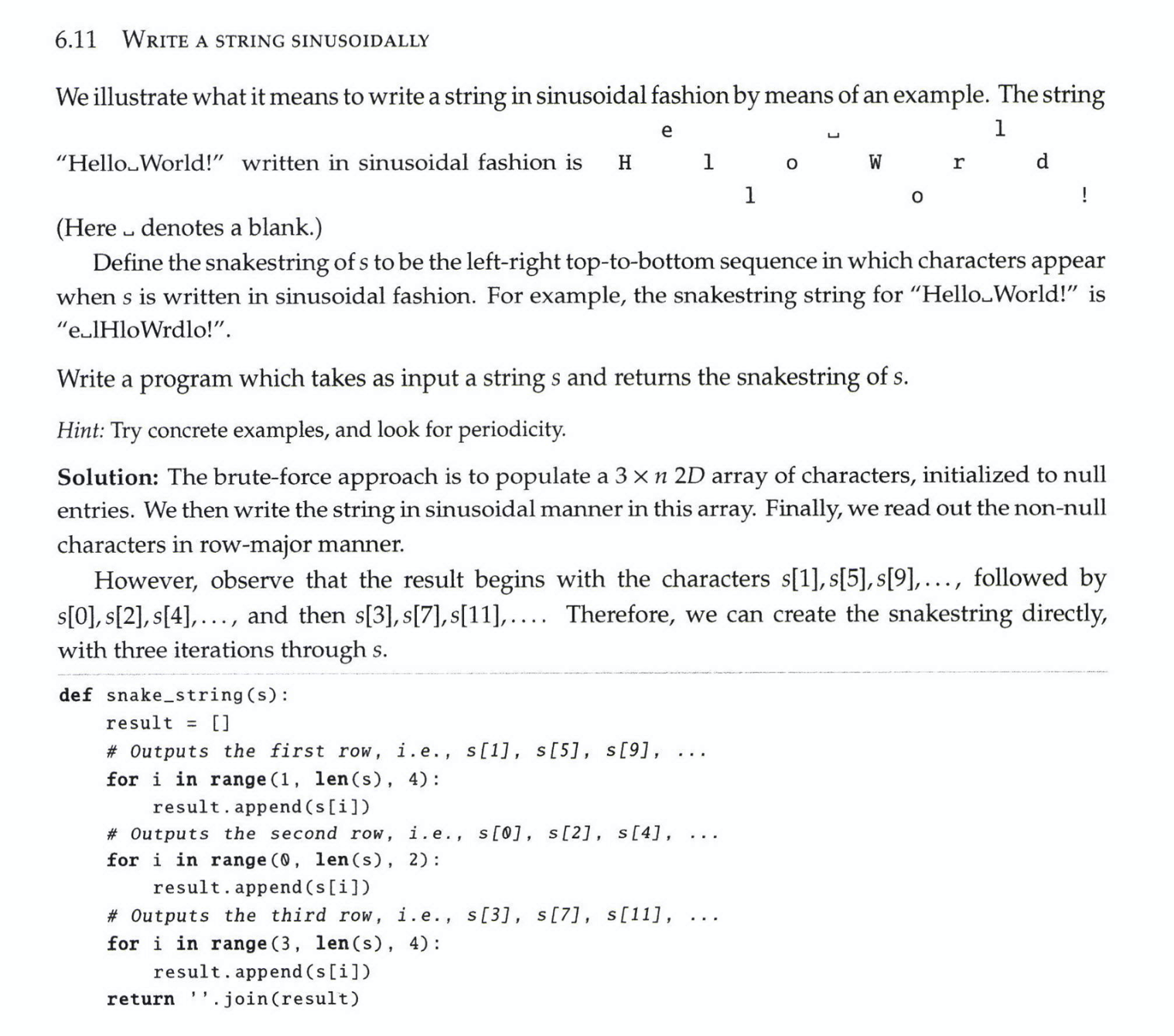• One Edit Distance *

"""
161. One Edit Distance

Given two strings s and t, return true if they are both one edit distance apart, otherwise return false.
A string s is said to be one distance apart from a string t if you can:
Insert exactly one character into s to get t.
Delete exactly one character from s to get t.
Replace exactly one character of s with a different character to get t.

Example 1:
Input: s = "ab", t = "acb"
Output: true
Explanation: We can insert 'c' into s to get t.
Example 2:
Input: s = "", t = ""
Output: false
Explanation: We cannot get t from s by only one step.
Example 3:
Input: s = "a", t = ""
Output: true
Example 4:
Input: s = "", t = "A"
Output: true

https://leetcode.com/problems/one-edit-distance

do https://leetcode.com/problems/edit-distance after this
"""

class Solution:

def isOneEditDistance(self, s: str, t: str):
# ensure one edit distance
if abs(len(t) - len(s)) > 1:
return False
if s == t:
return False
# ensure t is longer
if len(t) < len(s):
return self.isOneEditDistance(t, s)

edited = False
s_idx, t_idx = 0, 0
while s_idx < len(s) or t_idx < len(t):
# one of them has reached end
if s_idx == len(s) or t_idx == len(t):
if edited or t_idx == len(t):
return False
# can remove last element
return t_idx == len(t)-1

# same char
if s[s_idx] == t[t_idx]:
s_idx += 1
t_idx += 1

# try delete from t
elif self.is_valid_move(s, t, s_idx, t_idx+1) and not edited:
t_idx += 1
edited = True

# try replace
elif self.is_valid_move(s, t, s_idx+1, t_idx+1) and not edited:
s_idx += 1
t_idx += 1
edited = True
# try insert
# if we know len(t) is always longer or equal to len(s),
#   replaces and deletes will work
#   insert does the opposite of delete
else:
return False

return edited

def is_valid_move(self, s, t, s_idx, t_idx):
if s_idx == len(s) or t_idx == len(t):
return s_idx == len(s) and t_idx == len(t)

if s[s_idx] == t[t_idx]:
return True

return False

• Longest Palindromic Substring

"""
Longest Palindromic Substring:

Given a string s, return the longest palindromic substring in s.
https://leetcode.com/problems/longest-palindromic-substring/
https://www.algoexpert.io/questions/Longest%20Palindromic%20Substring
"""

class Solution:
def longestPalindrome(self, s: str):
if len(s) < 2:
return s

longest = idx = 1
left = right = 0
for idx in range(1, len(s)):

# check for both even and odd palindromes
# Examples: even -> zyaaaagh, odd -> zyaaagh
even, left_even, right_even = self.expandFromMiddle(s, idx-1, idx)
odd, left_odd, right_odd = self.expandFromMiddle(s, idx-1, idx+1)

# record the largest palindrome we found
if even > odd and even > longest:
longest = even
left = left_even
right = right_even
if odd > even and odd > longest:
longest = odd
left = left_odd
right = right_odd

return s[left:right+1]

def expandFromMiddle(self, s, left, right):
if right >= len(s) or s[left] != s[right]:
return 0, left, right

# pointers showing how far we have expanded (which marks how wide the palindrome is)
exp_left = left
exp_right = right

while left >= 0 and right < len(s) and s[left] == s[right]:
# expand
exp_left = left
exp_right = right

# move on to checking the next
left -= 1
right += 1

# return len of the longest palindrome we found
return ((exp_right - exp_left) + 1), exp_left, exp_right

• Break a Palindrome

LeetCode 1328 - Break a Palindrome

"""
1328. Break a Palindrome

Given a palindromic string of lowercase English letters palindrome, replace exactly one character with any lowercase English letter so that the resulting string is not a palindrome and that it is the lexicographically smallest one possible.
Return the resulting string. If there is no way to replace a character to make it not a palindrome, return an empty string.
A string a is lexicographically smaller than a string b (of the same length) if in the first position where a and b differ, a has a character strictly smaller than the corresponding character in b.
For example, "abcc" is lexicographically smaller than "abcd" because the first position they differ is at the fourth character, and 'c' is smaller than 'd'.

Example 1:
Input: palindrome = "abccba"
Output: "aaccba"
Explanation: There are many ways to make "abccba" not a palindrome, such as "zbccba", "aaccba", and "abacba".
Of all the ways, "aaccba" is the lexicographically smallest.
Example 2:
Input: palindrome = "a"
Output: ""
Explanation: There is no way to replace a single character to make "a" not a palindrome, so return an empty string.
Example 3:
Input: palindrome = "aa"
Output: "ab"
Example 4:
Input: palindrome = "aba"
Output: "abb"

https://leetcode.com/problems/break-a-palindrome
"""

class Solution:
def breakPalindrome(self, palindrome: str):
if len(palindrome) <= 1:
return ""

# replace first non-a
is_odd = len(palindrome) % 2 != 0
for idx, char in enumerate(palindrome):
if is_odd and idx == len(palindrome) // 2:
continue

if char != "a":
return palindrome[:idx] + "a" + palindrome[idx+1:]

# no valid non-a was found so replace the last character
# eg: aaa->aab, aaaa->aaab, aba->abb
return palindrome[:-1] + "b"

• String Rotation

"""
String Rotation:

Assume you have a method isSubstring which checks if one word is a substring of another.
Given two strings, sl and s2, write code to check if s2 is a rotation of sl using only one call to isSubstring
(e.g.,"waterbottle" is a rotation of"erbottlewat").
"""

def is_substring(str, substring):
return substring in str

def string_rotation(s1, s2):
if len(s1) != len(s2):
return False
return is_substring(s1+s1, s2)

• Group Shifted Strings *

"""
249. Group Shifted Strings

We can shift a string by shifting each of its letters to its successive letter.
For example, "abc" can be shifted to be "bcd".
We can keep shifting the string to form a sequence.
For example, we can keep shifting "abc" to form the sequence: "abc" -> "bcd" -> ... -> "xyz".
Given an array of strings strings, group all strings[i] that belong to the same shifting sequence. You may return the answer in any order.

Example 1:
Input: strings = ["abc","bcd","acef","xyz","az","ba","a","z"]
Output: [["acef"],["a","z"],["abc","bcd","xyz"],["az","ba"]]
Example 2:
Input: strings = ["a"]
Output: [["a"]]

https://leetcode.com/problems/group-shifted-strings
"""

import collections

class Solution:
def getMovement(self, string):
movement = []
for i in range(1, len(string)):
move = ord(string[i]) - ord(string[i-1])
if move < 0:  # alphabet is a closed loop
move += 26
movement.append(move)
return tuple(movement)

def groupStrings(self, strings):
moves = collections.defaultdict(list)

for string in strings:
moves[self.getMovement(string)].append(string)

return list(moves.values())

• Count and Say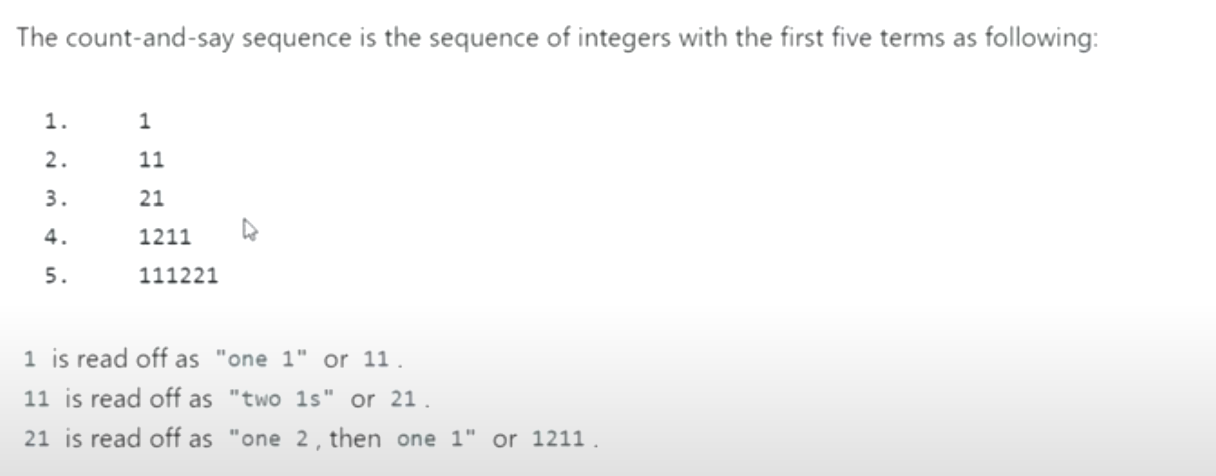"""
Count and Say:

The count-and-say sequence is a sequence of digit strings defined by the recursive formula:
countAndSay(1) = "1"
countAndSay(n) is the way you would "say" the digit string from countAndSay(n-1), which is then converted into a different digit string.
To determine how you "say" a digit string, split it into the minimal number of groups so that each group is a contiguous section all of the same character. Then for each group, say the number of characters, then say the character. To convert the saying into a digit string, replace the counts with a number and concatenate every saying.
For example, the saying and conversion for digit string "3322251":

Given a positive integer n, return the nth term of the count-and-say sequence.

Example 1:
Input: n = 1
Output: "1"
Explanation: This is the base case.
Example 2:
Input: n = 4
Output: "1211"
Explanation:
countAndSay(1) = "1"
countAndSay(2) = say "1" = one 1 = "11"
countAndSay(3) = say "11" = two 1's = "21"
countAndSay(4) = say "21" = one 2 + one 1 = "12" + "11" = "1211"

https://leetcode.com/problems/count-and-say
"""

class Solution:
def countAndSay(self, n: int):
if n == 1:
return '1'

res = 
for _ in range(n-1):
new_res = []

curr = res
count = 1
for idx in range(1, len(res)):
if res[idx] == curr:
count += 1
else:
new_res.append(count)
new_res.append(curr)
# reset
curr = res[idx]
count = 1

new_res.append(count)
new_res.append(curr)

res = new_res

return "".join([str(num) for num in res])

• Interconvert Strings & Integers

"""
Interconvert Strings & Integers:

A string is a sequence of characters. A string may encode an integer, e.g., "123" encodes 123.
In this problem, you are to implement methods that take a string representing an integer and return the corresponding integer, and vice versa.
Your code should handle negative integers. You cannot use library functions like int in Python.
Implement an integer to string conversion function, and a string to integer conversion function,
For example,
if the input to the first function is the integer 314,
it should return the string "314"
and if the input to the second function is the string "314" it should return the integer 314.

EPI 6.1
"""

"""
------------------------------------------------------------------------------------------------------------------------------------------------------------------------------------------------------------------
int to string
"""

def single_digit_to_char(digit):
return chr(ord('0')+digit)

def int_to_string(x: int):
if x == 0:
return "0"

is_neg = False
if x < 0:
is_neg, x = True, -x

result = []
while x > 0:
result.append(single_digit_to_char(x % 10))
x = x // 10

if is_neg:
result.append('-')
return "".join(reversed(result))

"""
------------------------------------------------------------------------------------------------------------------------------------------------------------------------------------------------------------------
string to int
"""

def single_char_to_int(character):
num_mapping = {
"1": 1,
"2": 2,
"3": 3,
"4": 4,
"5": 5,
"6": 6,
"7": 7,
"8": 8,
"9": 9,
"0": 0,
}
return num_mapping[character]

def string_to_int(s: str):

is_neg = False
start_idx = 0
if s and s == "-":
is_neg, start_idx = True, 1
if s and s == "+":
start_idx = 1

running_sum = 0
multiplier = 1

for idx in reversed(range(start_idx, len(s))):
running_sum += single_char_to_int(s[idx])*multiplier
multiplier *= 10

if is_neg:
return -running_sum
return running_sum

• Letter Case Permutations *

• Caesar Cipher Encryptor

"""
Caesar Cipher Encryptor:

Given a non-empty string of lowercase letters and a non-negative integer representing a key,
write a function that returns a new string obtained by shifting every letter in the input string by k positions in the alphabet,
where k is the key.
Note that letters should "wrap" around the alphabet;
in other words, the letter z shifted by one returns the letter a.

https://www.algoexpert.io/questions/Caesar%20Cipher%20Encryptor
"""

def caesarCipherEncryptor_(string, key):
key = key % 26  # handle large numbers
letters = []

for char in string:
moved_ord = ord(char) + key

if moved_ord > ord('z'):
moved_ord -= 26

letters.append(chr(moved_ord))

return "".join(letters)

• Minimum Window Substring **

Minimum Window Substring - Airbnb Interview Question - Leetcode 76

"""
76. Minimum Window Substring

Given two strings s and t of lengths m and n respectively,
return the minimum window substring of s such that every character in t (including duplicates) is included in the window.
If there is no such substring, return the empty string "".

The testcases will be generated such that the answer is unique.

A substring is a contiguous sequence of characters within the string.

Example 1:
Input: s = "ADOBECODEBANC", t = "ABC"
Output: "BANC"
Explanation: The minimum window substring "BANC" includes 'A', 'B', and 'C' from string t.
Example 2:
Input: s = "a", t = "a"
Output: "a"
Explanation: The entire string s is the minimum window.
Example 3:
Input: s = "a", t = "aa"
Output: ""
Explanation: Both 'a's from t must be included in the window.
Since the largest window of s only has one 'a', return empty string.

https://leetcode.com/problems/minimum-window-substring

Prerequisites:
- https://leetcode.com/problems/find-all-anagrams-in-a-string/ (https://www.notion.so/paulonteri/Sliding-Window-f6685a15f97a4ca2bb40111e2b264fb2#618e5fb94ea54bee8ff5eb7ab0c155ab)
- https://leetcode.com/problems/permutation-in-string
"""

import collections

""" BF """

class Solution_:

def minWindow(self, s: str, t: str):
t_count = collections.Counter(t)
store = collections.defaultdict(int)

idx = 0
while idx < len(s) and not self.hasAllInT(store, t_count):
store[s[idx]] += 1
idx += 1

if not self.hasAllInT(store, t_count):
return ""

res = [0, idx-1]
left = 0
right = idx-1
while left <= right and right < len(s):
# # we have all needed characters
if left <= right and self.hasAllInT(store, t_count):
# record size
res = min(res, [left, right], key=lambda x: x-x)

# reduce size
store[s[left]] -= 1
if store[s[left]] == 0:
store.pop(s[left])
left += 1

# # do not have all needed characters - expand window
else:
right += 1
if right < len(s):
store[s[right]] += 1

return s[res:res+1]

def hasAllInT(self, store, t_count):

for char in t_count:
if char not in store:
return False
if store[char] < t_count[char]:
return False
return True

""" Optimal """

class Solution:

def minWindow(self, s: str, t: str):
t_count = collections.Counter(t)

# # window
window_val_count = collections.defaultdict(int)  # default 0
num_of_valid_chars = self.increase_window(-1, s, t_count, window_val_count, 0)

res = (0, float("inf"))

left, right = 0, 0
while left <= right and right < len(s):

# we have all characters - decrease window
if num_of_valid_chars == len(t_count):
res = min(res, (left, right), key=lambda x: x-x)
num_of_valid_chars = self.decrease_window(
left, s, t_count, window_val_count, num_of_valid_chars)
left += 1

# do not have all characters - increase window
else:
num_of_valid_chars = self.increase_window(
right, s, t_count, window_val_count, num_of_valid_chars)
right += 1

if res == float('inf'):
return ""
return s[res:res+1]

def decrease_window(self, left, s, t_count, window_val_count, num_of_valid_chars):
left_char = s[left]
if left_char not in t_count:
return num_of_valid_chars

window_val_count[left_char] -= 1

# correct valid chars: one is now missing
if had_needed and window_val_count[left_char] < t_count[left_char]:
return num_of_valid_chars-1

return num_of_valid_chars

def increase_window(self, right, s, t_count, window_val_count, num_of_valid_chars):
if right+1 >= len(s):
return num_of_valid_chars

right_char = s[right+1]
if right_char not in t_count:
return num_of_valid_chars

window_val_count[right_char] += 1

# correct valid chars: one is now added
if not had_needed and window_val_count[right_char] >= t_count[right_char]:
return num_of_valid_chars+1

return num_of_valid_chars

• Minimum Characters For Words

"""
Minimum Characters For Words:

Write a function that takes in an array of words and returns the smallest array of characters needed to form all of the words.
The characters don't need to be in any particular order.
For example, the characters ["y", "r", "o", "u"] are needed to form the words ["your", "you", "or", "yo"].
Note: the input words won't contain any spaces; however, they might contain punctuation and/or special characters.

Sample Input
words = ["this", "that", "did", "deed", "them!", "a"]
Sample Output
["t", "t", "h", "i", "s", "a", "d", "d", "e", "e", "m", "!"]
// The characters could be ordered differently.

https://www.algoexpert.io/questions/Minimum%20Characters%20For%20Words
"""

from collections import defaultdict

# O(n) time | O(n) space - where n is the length of the word
def get_word_characters(word):
char_count = defaultdict(int)
for char in word:
char_count[char] += 1

return char_count

# O(n*l) time | O(c) space
# where n is the number of words, l the length of the longest word, c the number of unique charactters
def minimumCharactersForWords(words):
min_character_count = defaultdict(int)

for word in words:
word_char_count = get_word_characters(word)
for char in word_char_count:
# update count if we find a word that needs more
min_character_count[char] = max(
min_character_count[char], word_char_count[char]
)

# convert hash table to list
min_character_count_list = []
for char in min_character_count:
count = min_character_count[char]
while count > 0:
min_character_count_list.append(char)
count -= 1

return min_character_count_list

"""
- Draw Example(s)
- Test case
- Brute force
- Explain Solution / Algorithm
- Talk through edge cases
- Pseudocode
- Code

[
"this",  -> "t","h","i","s"
"that",  -> "t","t","h","a"
"did"    -> "d","d","i"
]

["t","t","h","i","s","d","d"]

- have an output array that will store the char count
- for each word, calculate the unique character count. Then check

def get_word_characters(word):
return {}

def minimumCharactersForWords(words):
min_character_count = {}
for word in words:
word_char_count = get_word_characters(word)
# coompare the word_char_count & min_character_count then update if necessary

return convert_to_list(min_character_count)

"""


"""

You're given a string of length 12 or smaller, containing only digits.
Write a function that returns all the possible IP addresses that can be created by inserting three .s in the string.
An IP address is a sequence of four positive integers that are separated by .s, where each individual integer is within the range 0 - 255, inclusive.
An IP address isn't valid if any of the individual integers contains leading 0s.
For example, "192.168.0.1" is a valid IP address, but "192.168.00.1" and "192.168.0.01" aren't, because they contain "00" and 01, respectively.
Another example of a valid IP address is "99.1.1.10"; conversely, "991.1.1.0" isn't valid, because "991" is greater than 255.
Your function should return the IP addresses in string format and in no particular order.
If no valid IP addresses can be created from the string, your function should return an empty list.

Sample Input
string = "1921680"
Sample Output
[
"1.9.216.80",
"1.92.16.80",
"1.92.168.0",
"19.2.16.80",
"19.2.168.0",
"19.21.6.80",
"19.21.68.0",
"19.216.8.0",
"192.1.6.80",
"192.1.68.0",
"192.16.8.0"
]
// The IP addresses could be ordered differently.
"""

def is_valid_ip(string):

string_as_num = int(string)
if string_as_num < 0 or string_as_num > 255:
return False

return len(str(string_as_num)) == len(string)

# O(1) time | O(1) space |-> there are a limited/finite/constant number (2^32) of IP Addresses
length = len(string)

ip_address = ['', '', '', '']
for i_one in range(1, min(length, 4)):
continue  # stop current for loop run

for i_two in range(i_one+1, min(length, i_one+4)):
continue  # stop current for loop run (for i_two)

for i_three in range(i_two+1, min(length, i_two+4)):


• Valid Palindrome II

"""
Valid Palindrome II:

Given a string s, return true if the s can be palindrome after deleting at most one character from it.

Example 1:
Input: s = "aba"
Output: true
Example 2:
Input: s = "abca"
Output: true
Explanation: You could delete the character 'c'.
Example 3:
Input: s = "abc"
Output: false

https://leetcode.com/problems/valid-palindrome-ii
"""

class Solution:
def validPalindrome(self, s: str):

left = 0
right = len(s)-1
while left < right:
# left and right are same
if s[left] == s[right]:
left += 1
right -= 1

# left and right not same
else:
return self.isPalindrome(s, left) or self.isPalindrome(s, right)

return True

def isPalindrome(self, s, skip):
# we will start be comparing the left most char & the right most char
a_pointer = 0
b_pointer = len(s) - 1

while a_pointer < b_pointer:
# skip
if a_pointer == skip:
a_pointer += 1
if b_pointer == skip:
b_pointer -= 1

# check if not the same
if s[a_pointer] != s[b_pointer]:
return False

# move on to the next set of chars
a_pointer += 1
b_pointer -= 1
return True

• Reverse Words in a String -

"""
Reverse Words in a String:

Given an input string s, reverse the order of the words.
A word is defined as a sequence of non-space characters.
The words in s will be separated by at least one space.
Return a string of the words in reverse order concatenated by a single space.
Note that s may contain leading or trailing spaces or multiple spaces between two words.
The returned string should only have a single space separating the words. Do not include any extra spaces.

Reverse Words In String:
Write a function that takes in a string of words separated by one or more whitespaces and returns a string that has these words in reverse order.
For example, given the string "tim is great", your function should return "great is tim".
For this problem, a word can contain special characters, punctuation, and numbers.
The words in the string will be separated by one or more whitespaces, and the reversed string must contain the same whitespaces as the original string.
For example, given the string "whitespaces 4" you would be expected to return "4 whitespaces".
Note that you're not allowed to to use any built-in split or reverse methods/functions. However, you are allowed to use a built-in join method/function.
Also note that the input string isn't guaranteed to always contain words.

Sample Input
string = "AlgoExpert is the best!"
Sample Output
"best! the is AlgoExpert"

https://leetcode.com/problems/reverse-words-in-a-string/
https://www.algoexpert.io/questions/Reverse%20Words%20In%20String
"""

# O(n) time | O(n) space - where n is the length of the string
def reverseWordsInString(string):
words = []

idx = len(string) - 1
while idx >= 0:
# white space
if string[idx] == " ":
words.append(" ")
idx -= 1

# words
else:
# get word's beginning
start = idx
while start - 1 >= 0 and string[start - 1] != " ":
start -= 1
# add word to words array
words.append(string[start:idx+1])

idx = start - 1
return "".join(words)

• Strobogrammatic Number

"""
Strobogrammatic Number

Given a string num which represents an integer, return true if num is a strobogrammatic number.
A strobogrammatic number is a number that looks the same when rotated 180 degrees (looked at upside down).

Example 1:
Input: num = "69"
Output: true
Example 2:
Input: num = "88"
Output: true
Example 3:
Input: num = "962"
Output: false
Example 4:
Input: num = "1"
Output: true

https://leetcode.com/problems/strobogrammatic-number
"""

class Solution:
def isStrobogrammatic(self, num):
rotated_digits = {'0': '0', '1': '1', '8': '8', '6': '9', '9': '6'}

rotated = list(num)
for idx, char in enumerate(rotated):
if char not in rotated_digits:
return False

rotated[idx] = rotated_digits[char]

rotated.reverse()
return "".join(rotated) == num

• Largest lexicographical string with at most K consecutive elements

screencapture-geeksforgeeks-org-largest-lexicographical-string-with-at-most-k-consecutive-elements-2021-10-22-12_21_18.pdf

• Multi String Search *

"""
Multi String Search:

Write a function that takes in a big string and an array of small strings, all of which are smaller in length than the big string.
The function should return an array of booleans, where each boolean represents whether the small string at that index in the array of small strings is contained in the big string.
Note that you can't use language-built-in string-matching methods.

Sample Input #1
bigString = "this is a big string"
smallStrings = ["this", "yo", "is", "a", "bigger", "string", "kappa"]
Sample Output #1
[true, false, true, true, false, true, false]
Sample Input #2
bigString = "abcdefghijklmnopqrstuvwxyz"
smallStrings = ["abc", "mnopqr", "wyz", "no", "e", "tuuv"]
Sample Output #2
[true, true, false, true, true, false]

https://www.algoexpert.io/questions/Multi%20String%20Search
"""

endSymbol = "*"

class Trie:
def __init__(self):
self.root = {}
self.endSymbol = endSymbol

current = self.root
for letter in word:
if letter not in current:
current[letter] = {}
current = current[letter]
current[self.endSymbol] = (word, idx)

def find_words(trie, bigString, found_words, idx):
# # validate
if idx == len(bigString) or bigString[idx] not in trie:
return
char = bigString[idx]
curr_trie = trie[char]

# record all words found
if endSymbol in curr_trie:
_, i = curr_trie[endSymbol]
found_words[i] = True

find_words(curr_trie, bigString, found_words, idx+1)

# O(ns + bs) time | O(ns) space
# b len(big), s len(small), n - no. of small strings,
def multiStringSearch(bigString, smallStrings):
found_words = [False] * len(smallStrings)

# # create trie
trie = Trie()
for idx, word in enumerate(smallStrings):

# # find words
for idx in range(len(bigString)):
find_words(trie.root, bigString, found_words, idx)

return found_words


## Questions to ask the interviewer

Is it case sensitive?

Are there special characters?

Is there whitespace? Is it significant?

## Tips

Try iterating form the end

## Basics

Functions

.count(str) returns how many times the str substring appears in the given string.

.upper() converts the string to uppercase.

.lower() converts the string to lowercase.

.swapcase()

.replace(old, new) replaces all occurrences of old with new.

.find(str)

.split()

.startswith(prefix)

.endswith(suffix)

.isalpha() returns true if a string only contains letters.

.isnumeric() returns true if all characters in a string are numbers.

.isalnum() only returns true if a string contains alphanumeric characters, without symbols.

Are similar to arrays

print(list(enumerate("cold")))  # [(0, 'c'), (1, 'o'), (2, 'l'), (3, 'd')]
print("expensive" not in "The best things in life are free!") # True


### Conversion to unicode

print(ord("0")) # 48

print(ord("a")) # 97
print(chr(97)) # a


### Convert single digit integer to unicode

print(chr( ord('0') + 2 )) # 9
# or
two_ord = ord('0') + 2
print(chr(two_ord)) # 9


### Change a string

Strings are immutable. This means that elements of a string cannot be changed once it has been assigned. We can simply reassign different strings to the same name.

string = "Hello world!"

string = "h"
# TypeError: 'str' object does not support item assignment

string = "hello world!"
print string
# hello world!


### Reverse

Need to convert string to list and then reverse().

string = "Hello world!"

string.reverse()
# AttributeError: 'str' object has no attribute 'reverse'

reversed(string) + "apple"
# TypeError: unsupported operand type(s) for +: 'reversed' and 'str'

print string[::-1]
# !dlrow olleH


### .upper() .lower() .swapcase()

print string.upper()
# HELLO WORLD!

print string.lower()
# hello world!

print string.swapcase()
# 'hELLO WORLD!'


### .replace()

replaces all occurrences of old with new

str1 = "emre.me.emre.me"

print(str1.replace(".", "-"))
# emre-me-emre-me


### .count()

p = "paul is apaul is paul"

p.count("paul")
# 3


### .join()

x = ["", "2", "", "3"]

"".join(x) # '23'


### Slicing *

A subset of array slicing

word = "0123456789"

print(word) # 8
print(word[-2]) # 8

# Simple slicing
print(word[2:4]) # 23
print(word[2:]) # 23456789
print(word[:4]) # 0123 -> first 4

# reverse
print(word[2:8]) # 23456
print(word[2:-2]) # 23456

# stride
print(word[2:8:2]) # 246
print(word[::2]) # 02468
print(word[::1]) # 0123456789
print(word[::-2]) # 97531
print(word[::-1]) # 9876543210


### Sort section of an array using slicing

def sort(self, nums, sort_start):
nums[sort_start:] = list(sorted(nums[sort_start:]))


### Time complexity of Arrays,sort(String [])

• Given: String[] str, for example: str = {"Hello", "World", "John", "Doe"}
• Let n be the number of elements in the str array.
• Let m be the average/maximum # of characters for the strings in the str array
• Then, calling Arrays.sort(str) on this array would have the performance characteristic of O(m * n log n).

The reason it's O(m*n logn) is that the sorting algorithm itself will run O(n logn) comparison operations in order to perform the sort. And each comparison operation takes O(m) time to finish.

Though that is very much the worst-case scenario: Given, say, Hello and World, even though m = 5 for those two, the comparison completes after only 1 step; the algorithm compares H and W and answers immediately, never even looking at ello or orld. But if the strings are Hello1, Hello2, Hello3 and Hello4, then that's a guaranteed 5 'steps' for every time 2 strings are compared, and there will be O(n logn) comparisons.

If we use a naive sorting algorithm of O(N^2) time, then the total will be O(M * N^2)

### Note:

As said above, strings behave much like normal arrays, with the main distinction being that, strings are immutable, meaning that they can't be edited after creation. This also means that simple operations like appending a character to a string are more expensive than they might appear. The canonical example of an operation that's deceptively expensive due to string immutability is the following:

string = "this is a string"
newString = ""

for character in string:
newString += character


The operation above has a time complexity of O(n^2) where n is the length of string, because each addition of a character to newString creates an entirely new string and is itself an O(n) operation. Therefore, n O(n) operations are performed, leading to an O(n^2) time-complexity operation overall.

## StringBuilder

Imagine you were concatenating a list of strings. What would the running time of this code be? For simplicity, assume that the strings are all the same length (call this x) and that there are n strings.

On each concatenation,a new copy of the string is created, and the two strings are copied over,character by character. The first iteration requires us to copy x characters. The second iteration requires copying 2x characters. The third iteration requires 3x,and so on. The total time therefore is O(x + 2x + . . . + nx). This reduces to O(xn^2).

Why is it O(xn^2)? Because 1 + 2 + ... + n equals n(n+1)/2, or O(n^2)

StringBuilder can help you avoid.this problem. StringBuilder simply creates a resizable array of all the strings, copying them back to a string only when necessary.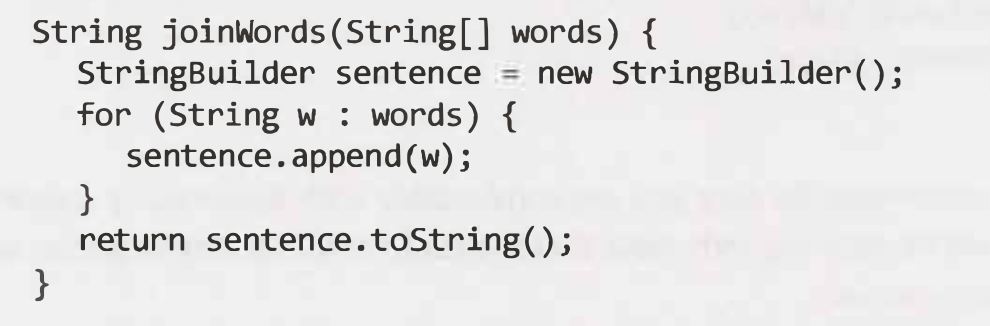StringBuilder (Java)

# Arrays

Python List

### Examples

Hare & Tortoise Algorithm

• Contains Duplicate

"""
Contains Duplicate

Given an integer array nums,
return true if any value appears at least twice in the array,
and return false if every element is distinct.

https://leetcode.com/problems/contains-duplicate/
"""

class Solution:
def containsDuplicate(self, nums):

store = set()

for num in nums:
# we have seen num before
if num in store:
return True
# record that we have just seen num

return False

def containsDuplicate_(self, nums):
return not len(set(nums)) == len(nums)

class Solution_:
def containsDuplicate(self, nums):
nums.sort()

for idx in range(1, len(nums)):
if nums[idx] == nums[idx-1]:
return True

return False

• When given problems (involving choice) with two arrays, where their indices represent an entity, you can recurse by iterating through each index deciding to include it or not

• 0/1 Knapsack
• Equal Subset Sum Partition

• Permutations *

• Letter Case Permutations *

• Pairs with Specific Difference *

"""
Pairs with Specific Difference:

Given an array arr of distinct integers and a nonnegative integer k,
write a function findPairsWithGivenDifference that returns an array of all pairs [x,y] in arr,
such that x - y = k. If no such pairs exist, return an empty array.

Note: the order of the pairs in the output array should maintain the order of the y element in the original array.

Examples:
[4,1], 3
[[4,1]]

[0,-1,-2,2,1], 1
[[1, 0], [0, -1], [-1, -2], [2, 1]]

[1,5,11,7], 4
[[5,1], [11,7]]

[1,5,11,7], 6
[[7,1],[11,5]]

https://www.pramp.com/challenge/XdMZJgZoAnFXqwjJwnpZ

Similar: https://leetcode.com/problems/k-diff-pairs-in-an-array/
"""

"""

curr - next = k
curr = k + next
"""

def find_pairs_with_given_difference(arr, k):
res = []
store = set(arr)

for num in arr:
if num+k in store:
res.append([num+k, num])

return res

"""
Works but doesn't obey ordering

curr - next = k
next = curr - k

def find_pairs_with_given_difference(arr, k):
res = []
store = set(arr)

for num in arr:
if num-k in store:
res.append([num, num-k])

return res

"""

print(find_pairs_with_given_difference(
[0, -1, -2, 2, 1], 1), "required: ", [[1, 0], [0, -1], [-1, -2], [2, 1]])
print(find_pairs_with_given_difference(
[1, 5, 11, 7], 4), "required: ", [[5, 1], [11, 7]])
print(find_pairs_with_given_difference(
[1, 5, 11, 7], 6), "required: ",  [[7, 1], [11, 5]])

• Three Sum *

• Four sum

4SUM (Leetcode) - Code & Whiteboard

4 Sum Problem | Leetcode #18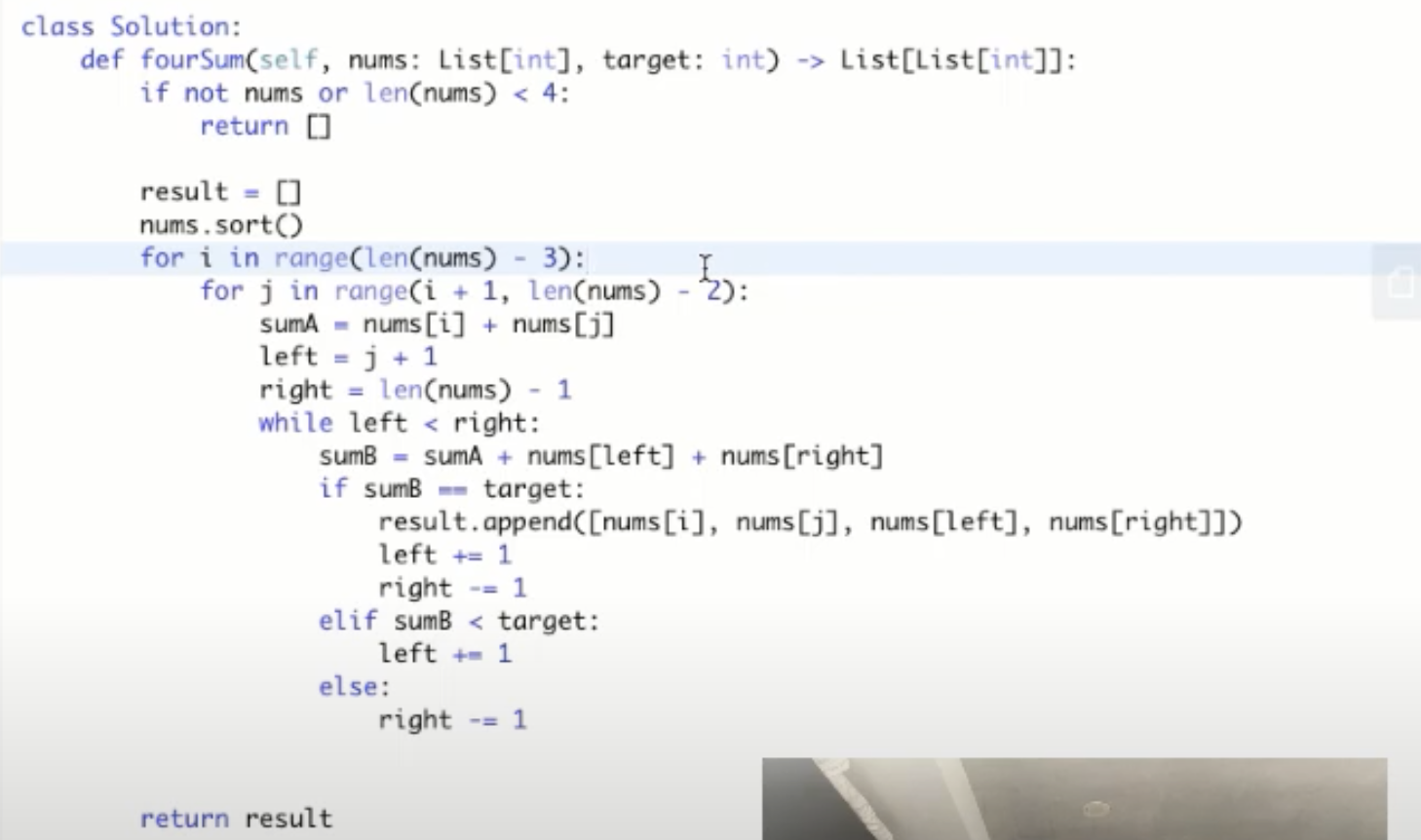If ignoring duplicates

https://youtu.be/29LH8QeJMzw

"""
4Sum:

Given an array nums of n integers, return an array of all the unique quadruplets [nums[a], nums[b], nums[c], nums[d]] such that:
0 <= a, b, c, d < n
a, b, c, and d are distinct.
nums[a] + nums[b] + nums[c] + nums[d] == target
You may return the answer in any order.

Example 1:
Input: nums = [1,0,-1,0,-2,2], target = 0
Output: [[-2,-1,1,2],[-2,0,0,2],[-1,0,0,1]]
Example 2:
Input: nums = [2,2,2,2,2], target = 8
Output: [[2,2,2,2]]
"""

"""
[1,0,-1,0,-2,2]

[-2, -1, 0, 0, 1, 2]

- four sum is a combination of two two_sums

- sort the input array so that we can skip duplicates

- have two loops with to iterate through all the possible two number combinations:
- for the rest of the numbers: find a two sum that = target - (arr[idx_loop_one] + arr[idx_loop_two])

"""

class Solution:

def fourSum(self, nums, target):
res = []
nums.sort()

for one in range(len(nums)):
if one > 0 and nums[one] == nums[one-1]:
continue  # skip duplicates
for two in range(one+1, len(nums)):
if two > one+1 and nums[two] == nums[two-1]:
continue  # skip duplicates

# # two sum
needed = target - (nums[one] + nums[two])
left = two + 1
right = len(nums)-1
while left < right:
# skip duplicates
if left > two + 1 and nums[left] == nums[left-1]:
left += 1
continue
if right < len(nums)-1 and nums[right] == nums[right+1]:
right -= 1
continue

total = nums[left] + nums[right]
if total < needed:
left += 1
elif total > needed:
right -= 1
else:
res.append(
[nums[one], nums[two], nums[left], nums[right]])
left += 1
right -= 1

return res


• Maximum Product Subarray **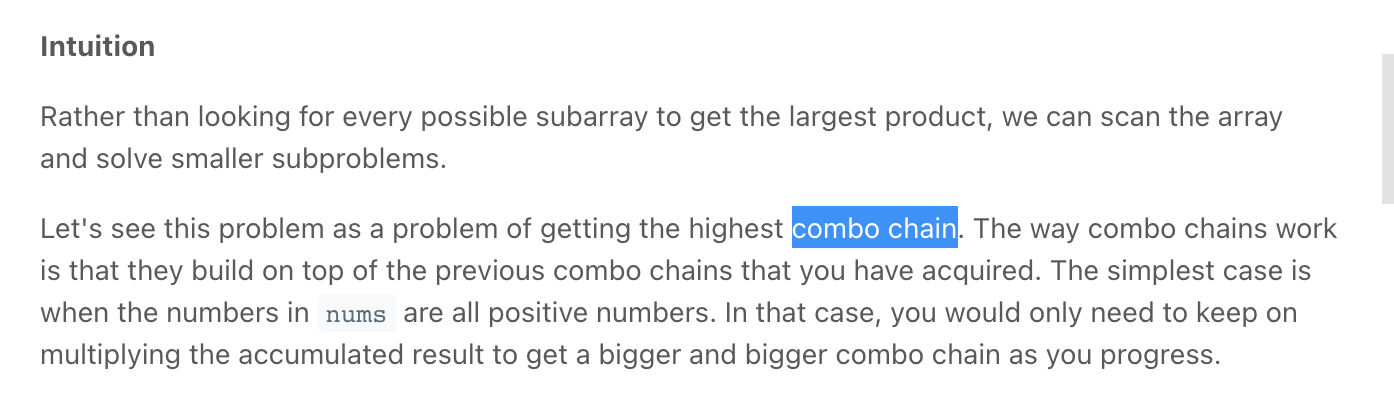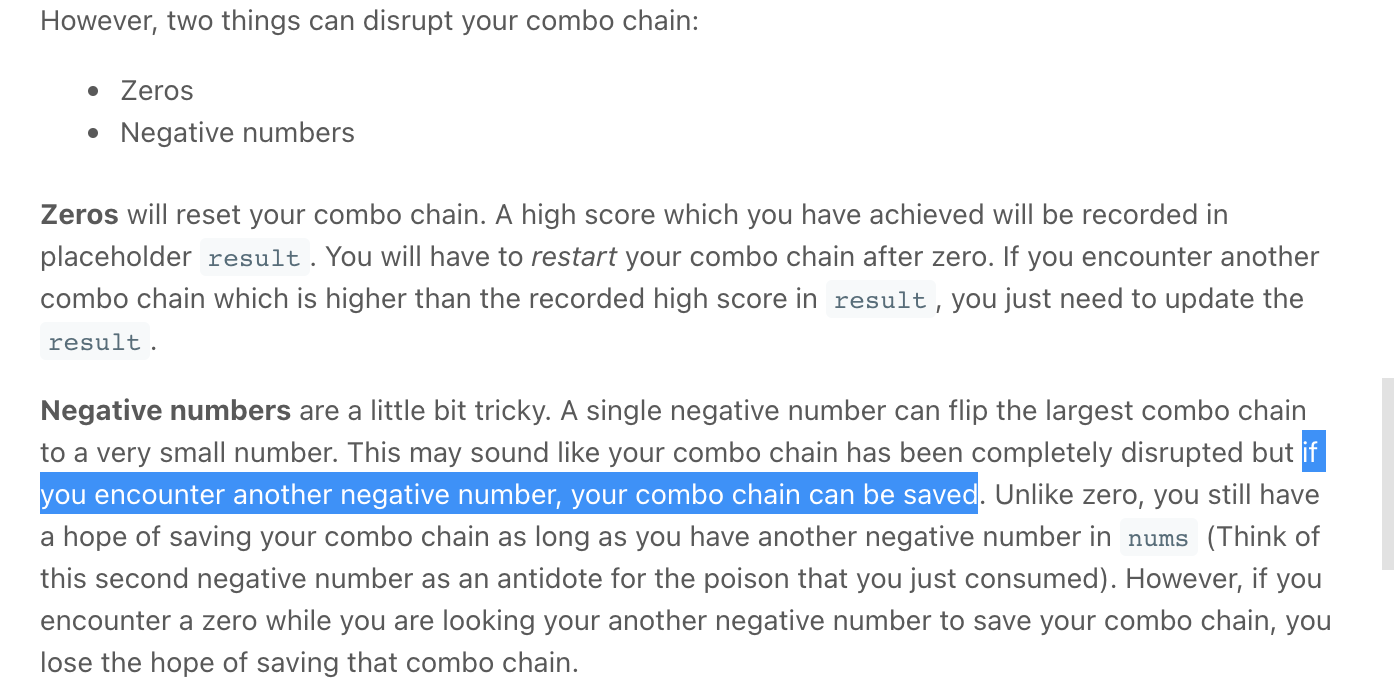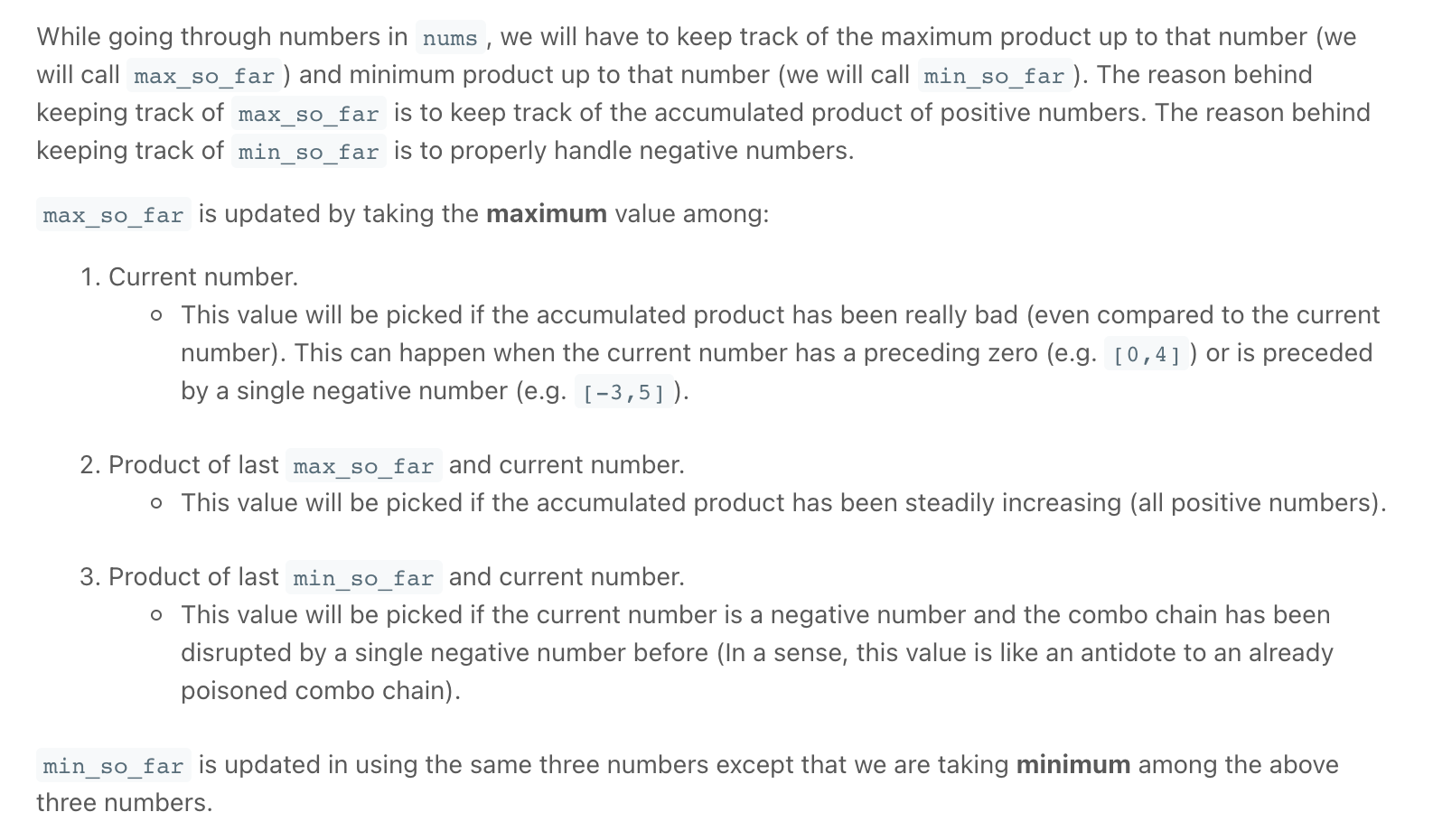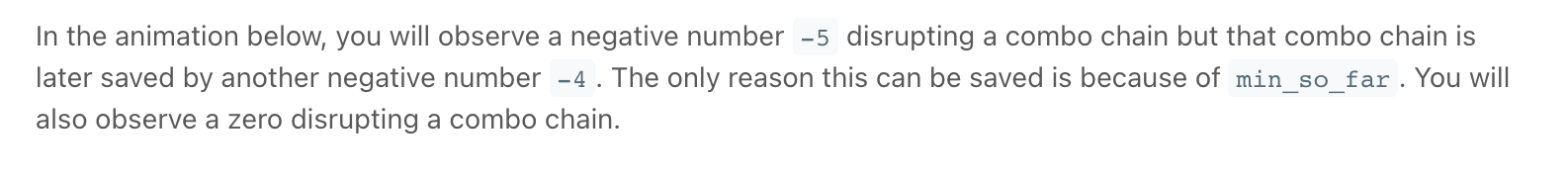Screen Recording 2021-10-16 at 21.05.00.mov

"""
Maximum Product Subarray:

Given an integer array nums, find the contiguous subarray within an array (containing at least one number) which has the largest product.
Example 1:
Input: [2,3,-2,4]
Output: 6
Explanation: [2,3] has the largest product 6.
Example 2:
Input: [-2,0,-1]
Output: 0
Explanation: The result cannot be 2, because [-2,-1] is not a subarray.

https://leetcode.com/problems/maximum-product-subarray/
"""

# O(n) time | O(1) space
class Solution:
def maxProduct(self, array):

if not array:
return -1

max_product = curr_max = curr_min = array

for idx in range(1, len(array)):

temp_curr_max = curr_max
curr_max = max(
curr_max * array[idx],
curr_min * array[idx],  # if array[idx] is negative [-2, 3, -4]
array[idx]  # helps if array[idx-1] is 0 eg: [0, 2]
)
curr_min = min(
temp_curr_max * array[idx],
curr_min * array[idx],
array[idx]
)

max_product = max(max_product, curr_max)

return max_product

sol = Solution()
print(sol.maxProduct([2, 2, 2, 1, -1, 5, 5]))
print(sol.maxProduct([-2, 3, -4]))
print(sol.maxProduct())
print(sol.maxProduct([]))
print(sol.maxProduct([-5]))
print(sol.maxProduct([0, 2, 2, 2, 1, -1, -5, -5]))
print(sol.maxProduct([0, 2]))

"""
Dynamic Programming:

Imagine that we have both max_prod[i] and min_prod[i] i.e. max product ending at i and min product ending at i.

Now if we have a negative number at arr[i+1] and if min_prod[i] is negative,
then the product of the two will be positive and can potentially be the largest product.
So, the key point here is to maintain both the max_prod and min_prod such that at iteration i, they refer to the max and min product ending at index i-1.

In short, One can have three options to make at any position in the array.
- You can get the maximum product by multiplying the current element with the maximum product calculated so far. (might work when current
element is positive).
- You can get the maximum product by multiplying the current element with minimum product calculated so far. (might work when current
element is negative).
- The current element might be a starting position for maximum product subarray.
Solution Steps

Initialize maxProduct with arr to store the maximum product so far.
Initialize to variables imax and imin to store the maximum and minimum product till i .
Iterate over the arr and for each negative element of arr, swap imax and imin. (Why?)
Update imax and imin as discussed above and finally return maxProduct
"""

• Maximum Subarray

"""
Maximum Subarray:

Given an integer array nums,
find the contiguous subarray (containing at least one number) which has the largest sum and return its sum.

Example 1:
Input: nums = [-2,1,-3,4,-1,2,1,-5,4]
Output: 6
Explanation: [4,-1,2,1] has the largest sum = 6.
Example 2:
Input: nums = 
Output: 1
Example 3:
Input: nums = [5,4,-1,7,8]
Output: 23

https://leetcode.com/problems/maximum-subarray/
"""

# O(n) time | O(1) space
class Solution:
def maxSubArray(self, nums):
# # # find the maximum subarray per given element:
# # check which one is larger:
# # adding the element to the current subarray or starting a new subarray at the element

# the max subarray we found's sum
max_sum = float("-inf")

# sum of the current subarray that we are working with
curr_subarray = float("-inf")
for num in nums:

# check if adding the num to the current subarray will be a larger sum than starting a new subarray at the element
# then the current subarray should be the longer/larger of the two
else:
curr_subarray = num

# record the largest (sum) we found
max_sum = max(max_sum, curr_subarray)

return max_sum

• Maximum Subarray *

• Subarray Sum Equals K *

LeetCode Subarray Sum Equals K Solution Explained - Java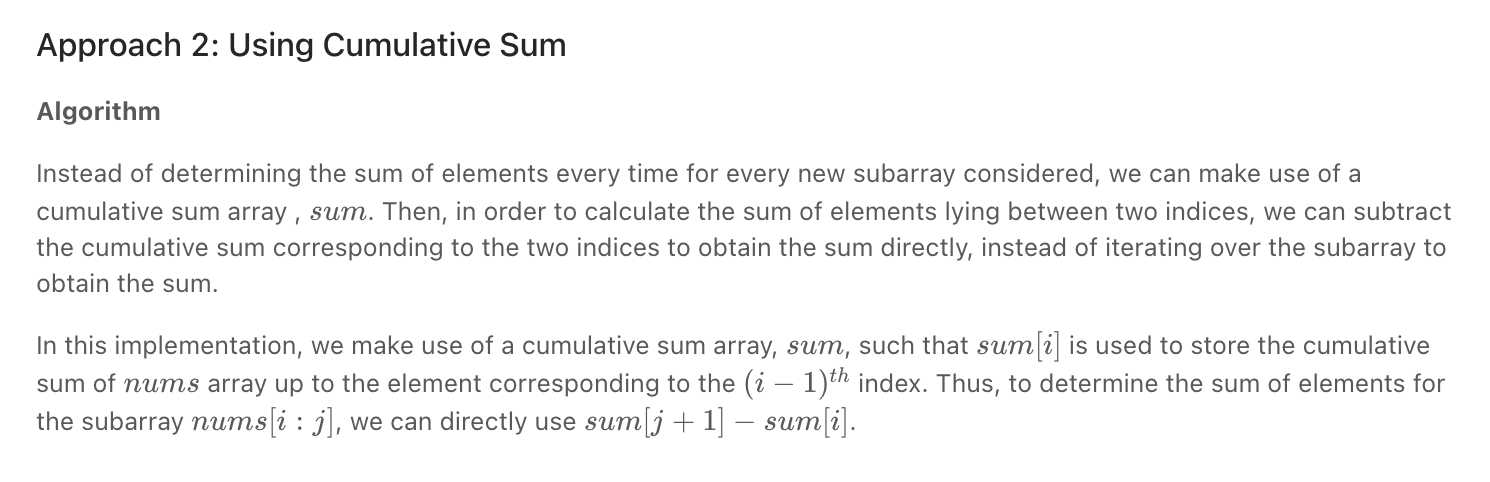"""
Subarray Sum Equals K

Given an array of integers and an integer k,
you need to find the total number of continuous subarrays whose sum equals to k.
Example 1:
Input: nums = [1,1,1], k = 2
Output: 2
Example 2:
Input: nums = [1,2,3], k = 3
Output: 2

https://leetcode.com/problems/subarray-sum-equals-k/
Do Next:
- https://leetcode.com/problems/path-sum-iii/
- https://leetcode.com/problems/continuous-subarray-sum
"""

from collections import defaultdict
from typing import List

"""

The idea behind the approach below is as follows: If the cumulative sum(represented by sum[i] for sum up to i^th index) up to two indices is the same,
the sum of the elements lying in between those indices is zero.
Extending the same thought further,
if the cumulative sum up to two indices, say i and j is at a difference of k
i.e. if sum[i] - sum[j] = k,
the sum of elements lying between indices i and j is k.

If you were at a current sum,
and you have seen (sum - k) before, it mean that,
we've seen an array of size k: - the distance between those two points is of size k
"""

# O(n) time | O(n) space | n = len(array)
class Solution:
# check if a total sum in the past plus the current will be equal to k
# if a (the current sum - a previous sum = k),
# then elements in the array between must add up to k
def subarraySum(self, nums: List[int], k: int):
res = 0

prev_sums = defaultdict(int)
# the store has to have 0 to deal with the case where k is in the list
prev_sums = 1
curr_sum = 0
# if (the current sum - a sum in the past = k),
# then the subarray in between them adds up to k
for num in nums:

curr_sum += num
needed_diff = curr_sum - k

# check for the needed_diff
if needed_diff in prev_sums:
# we add the number of possible times we can get that needed_diff
res += prev_sums[needed_diff]

# add the current sum to the store
prev_sums[curr_sum] += 1

return res

"""

# only works for arrays with positive integers
class Solution0:
def subarraySum(self, nums: List[int], k: int):
res = 0
total = nums

start = end = 0
while start < len(nums):
if total == k:
res += 1

# # edge cases
# when we cannot increase end or start
if end == len(nums)-1 or start == len(nums)-1:
total -= nums[start]
start += 1
continue

if total <= k:
end += 1
total += nums[end]
else:
total -= nums[start]
start += 1

return res

"""

• Continuous Subarray Sum ***

Complete explanation | mathematical | code with proper comments | O(N) | continuous subarray sum - LeetCode Discuss

Math behind the solutions - LeetCode Discuss

Python Solution with explanation - LeetCode Discuss

LeetCode 523. Continuous Subarray Sum Explanation and Solution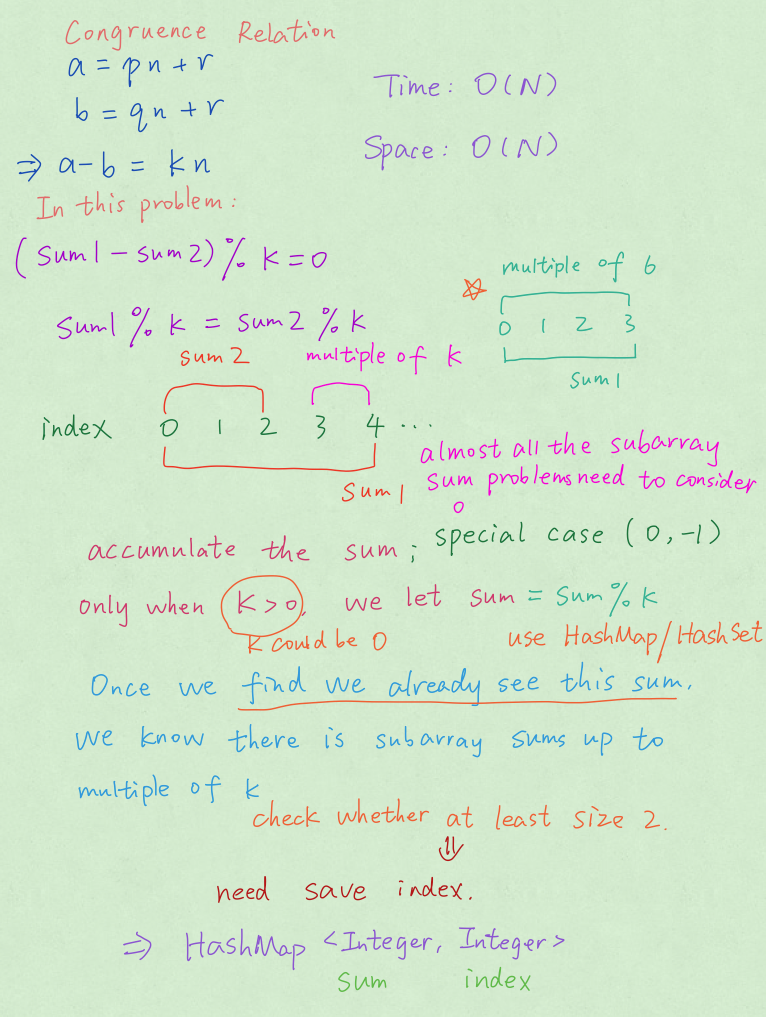"""

[1, 2, 3, 4,]
[1, 3, 6, 10]
10-1 =  19  = 2+3+4
6-1  =   5  = 2+3

if we store the cumulative sum for every point (idx) in the array,
if (sum2-sum1) % k = 0
then the numbers between sum2-sum1 add up to a multiple of k

Remember, there's another aspect to this problem. The subarray must have a minimum size of 2.
"""

class SolutionBF:
def checkSubarraySum(self, nums, k):
if len(nums) < 2:
return False

# 0: -1 is for edge case that current sum mod k == 0
# for when the current running sum is cleanly divisible by k
# e.g: nums = [4, 2], k = 3
sums = {0: -1}  # 0
cumulative_sum = 0
for idx, num in enumerate(nums):
cumulative_sum += num

for prev_sum in sums:
if (cumulative_sum-prev_sum) % k == 0 and idx-sums[prev_sum] >= 2:
return True

# if current sum mod k not in dict, store it so as to ensure the further values stay
if cumulative_sum not in sums:
sums[cumulative_sum] = idx

return False

"""
[1, 2, 3, 4,]  <= array
[1, 3, 6, 10] <= cumulative sums
10 -1 =  19  = 2+3+4
6 -1  =   5  = 2+3

if we store the cumulative sum for every point (idx) in the array,
if (sum2-sum1) % k = 0
then the numbers between sum2-sum1 add up to a multiple of k

if you find duplicated sum%k values, then that the sub array between those two indexes will actually be the solution.

---

eg: [15,10,10], k = 10
15%10 = 5
25%10 = 5
35%10 = 5

Did you realize something? No
Let's see- the sums 15 and 25 are giving the remainder 5 when divided by 10 and the difference between 15 and 25 i.;e. 10 is divisible by 10.
Let's check with sums 15 and 35 , both giving the remainder 5 but their difference is divisible by 10.

---

eg: [23, 2, 4, 6, 7], k = 6
[23,25,29, ...]

23%6 = 5
25%6 = 1
29%6 = 5

The cumulative sums 23 and 29 have the same remainder,
and their difference is a multiple of 6 (6 (2+4))

Why is getting 5 twice significant here?
Given any number, let's say 11 and another number 5.
11%5 = 1

After adding how much to 11 would we get remainder with 5 equal to 1 again?
In order to repeat a remainder you need to add the number you are dividing by, in this case 5.

if 11%5 = 1
then (11+5)%5 = 1
(11+10)%5 = 1 and so on

Therefore as in the above example, because we saw 5 as remainder repeat, that means that,
there was a cumulative sum somewhere in the list that added up to 6 (number we are dividing by).
That's why we would return true.

---

If two sums A and B are giving the same remainder when divided by a number K, then their difference abs(A-B) is always divisible by K.
Simply, If you get the same remainder again, it means that you've encountered some sum which is a multiple of K.
- Their difference is a multiple of k, that's why they have the same remainder

(sum2-sum1) % k = 0
sum2%k - sum1%k = 0
sum2%k = sum1%k

https://leetcode.com/problems/continuous-subarray-sum/discuss/688125/Lehman-explanation-of-the-math
https://leetcode.com/problems/continuous-subarray-sum/discuss/338417/Python-Solution-with-explanation

Remember, there's another aspect to this problem. The subarray must have a minimum size of 2.
"""

class Solution:
def checkSubarraySum(self, nums, k):
if len(nums) < 2:
return False

# 0: -1 is for edge case that current sum mod k == 0
# for when the current running sum is cleanly divisible by k
# e.g: nums = [4, 2], k = 3
sums = {0: -1}  # 0
cumulative_sum = 0
for idx, num in enumerate(nums):
cumulative_sum += num
remainder = cumulative_sum % k

# if current_sum mod k is in dict and index idx - sums[remainder] > 1, we got the Subarray!
# we use 2 not 1 because the element at sums[remainder] is not in the subarray we are talking about
if remainder in sums and idx - sums[remainder] >= 2:
return True

# if current sum mod k not in dict, store it so as to ensure the further values stay
if remainder not in sums:
sums[remainder] = idx

• Longest Consecutive Sequence *

"""
Longest Consecutive Sequence:

Given an unsorted array of integers nums, return the length of the longest consecutive elements sequence.
You must write an algorithm that runs in O(n) time.

Example 1:
Input: nums = [100,4,200,1,3,2]
Output: 4
Explanation: The longest consecutive elements sequence is [1, 2, 3, 4]. Therefore its length is 4.
Example 2:
Input: nums = [0,3,7,2,5,8,4,6,0,1]
Output: 9

Constraints:
0 <= nums.length <= 105
-109 <= nums[i] <= 109

https://www.algoexpert.io/questions/Largest%20Range
https://leetcode.com/problems/longest-consecutive-sequence/
"""
"""
Largest Range:

Write a function that takes in an array of integers and
returns an array of length 2 representing the largest range of integers contained in that array.
The first number in the output array should be the first number in the range,
while the second number should be the last number in the range.
A range of numbers is defined as a set of numbers that come right after each other in the set of real integers.
For instance, the output array [2, 6] represents the range {2, 3, 4, 5, 6}, which is a range of length 5.
Note that numbers don't need to be sorted or adjacent in the input array in order to form a range.
You can assume that there will only be one largest range.
Sample Input
array = [1, 11, 3, 0, 15, 5, 2, 4, 10, 7, 12, 6]
Sample Output
[0, 7]
"""

# O(nlog(n)) time
def largestRange(nums):
if len(nums) < 1:
return []

nums.sort()
res = [0, 0]

idx = 0
while idx < len(nums) - 1:
# check if start of consecutive nums
if not (nums[idx]+1 == nums[idx+1] or nums[idx] == nums[idx+1]):
idx += 1
continue

# find the numbers
end = idx+1
while end < len(nums)-1 and (nums[end]+1 == nums[end+1] or nums[end] == nums[end+1]):
end += 1

# record
res = max(res, [idx, end], key=lambda x: nums[x] - nums[x])

# move pointer
idx = end

return [nums[res], nums[res]]

"""
------------------------------------------------------------------------------------------------------------------------------------------------

- for each number try to build the largest number range from the input array
- the numbers can be stored in a set to improve lookup time

- for each num, if num-1 is in the set:
- do not check the num because it will be in num-1's range

"""

# O(n) time
class Solution:
def longestConsecutive(self, nums):
longest = 0

store = set(nums)

for num in store:
if num-1 in store:
# do not check the num because it will be in num-1's range
continue

# try to build the largest consecutive sequence from the input array
count = 1
while num+1 in store:
count += 1
num += 1

longest = max(count, longest)

return longest

• Max Subset Sum No Adjacent *

"""

Write a function that takes in an array of positive integers and
returns the maximum sum of non-adjacent elements in the array.
If the input array is empty, the function should return 0.

"""

return bfHelper(array, float('inf'), 0, 0)

if idx >= len(array):
return curr_sum

not_chosen = bfHelper(array, last_added, curr_sum, idx+1)  # ignore idx
chosen = -1
if last_added != idx-1:  # choose idx
chosen = bfHelper(array, idx, curr_sum + array[idx], idx+1)

return max(
not_chosen,
chosen
)

# ------------------------------------------------------------------------------------------------------------------------

"""
array: [7, 10, 12,          7,    9,    14]
max_sum_at_pos:  [7, 10, 19,         19,   28,    33]
[7, 10, 12+7,  10+7/19, 19+9, 19+14]

array: [30, 25, 50, 55, 100, 120]
max_sum_at_pos: [30, 30, 80, 80, 180, 200]

max_sums[i] = max(
(max_sums[i-1]),            -> i cannot be included
(max_sums[i-2] + array[i])  -> i can be included
)
"""

# 0(n) time | 0(n) space

if len(array) == 0:
return 0

# for each index in the input array,
#  find the maximum possible sum and store it in the max_sums array
# max_sums[i] = max( (max_sums[i-1]), (max_sums[i-2] + array[i]) )
max_sums = [array]
for idx in range(1, len(array)):

if idx == 1:
max_sums.append(max(array, array))
continue

prev_maxsum = max_sums[idx-1]
curr_plus_before_prev_maxsum = array[idx] + max_sums[idx-2]

# maximum sum at index
max_sums.append(
max(
curr_plus_before_prev_maxsum,
prev_maxsum)
)

return max_sums[-1]

# ------------------------------------------------------------------------------------------------------------------------

# 0(n) time | 0(1) space

if len(array) == 0:
return 0
elif len(array) == 1:
return array

prev_max = array
curr_max = max(array, array)  # at index 1
# for each index in the input array,
#  find the maximum possible sum and store it in the max_sums array
# max sum for array[i] = max( (curr_max), (array[idx] + prev_max) )
for idx in range(2, len(array)):

curr_plus_prevmax = array[idx] + prev_max

prev_max = curr_max
curr_max = max(curr_plus_prevmax, curr_max)

return curr_max

• Subarrays with Product Less than a Target *

• Array Of Products / Product of Array Except Self

"""
Array Of Products:
Product of Array Except Self:

Write a function that takes in a non-empty array of integers and returns an array of the same length,
where each element in the output array is equal to the product of every other number in the input array.
In other words, the value at output[i] is equal to the product of every number in the input array other than input[i].

Note that you're expected to solve this problem without using division.

https://www.algoexpert.io/questions/Array%20Of%20Products
https://leetcode.com/problems/product-of-array-except-self/
"""

# O(n) time | O(n) space - where n is the length of the input array (O(3n) time)
def arrayOfProducts(array):
res = array[:]

# We know that for each element, the product of all other elements
#  will be equal to the the products of the elements to its right and and the products of the elements to its left
# we can try to calculate that beforehand

# multiply left & right products for each element
left_products = *len(array)
running_left = 1  # first element will have a product of 1
for idx in range(len(array)):
left_products[idx] = running_left
running_left = array[idx] * running_left

# calculate products to the right of elements
right_products = *len(array)
running_right = 1  # last element will have a product of 1
for idx in reversed(range(len(array))):
right_products[idx] = running_right
running_right = array[idx] * running_right

# multiply left & right products for each element
for idx in range(len(array)):
res[idx] = left_products[idx] * right_products[idx]

return res

y = [5, 1, 4, 2]
x = [1, 2, 3, 4, 5]
print(arrayOfProducts(x))
print(arrayOfProducts(y))

• Array Of Products / Product of Array Except Self

"""
Array Of Products:
Product of Array Except Self:

Write a function that takes in a non-empty array of integers and returns an array of the same length,
where each element in the output array is equal to the product of every other number in the input array.
In other words, the value at output[i] is equal to the product of every number in the input array other than input[i].

Note that you're expected to solve this problem without using division.

https://www.algoexpert.io/questions/Array%20Of%20Products
https://leetcode.com/problems/product-of-array-except-self/
"""

# O(n) time | O(n) space - where n is the length of the input array (O(3n) time)
def arrayOfProducts(array):
res = array[:]

# We know that for each element, the product of all other elements
#  will be equal to the the products of the elements to its right and and the products of the elements to its left
# we can try to calculate that beforehand

# multiply left & right products for each element
left_products = *len(array)
running_left = 1  # first element will have a product of 1
for idx in range(len(array)):
left_products[idx] = running_left
running_left = array[idx] * running_left

# calculate products to the right of elements
right_products = *len(array)
running_right = 1  # last element will have a product of 1
for idx in reversed(range(len(array))):
right_products[idx] = running_right
running_right = array[idx] * running_right

# multiply left & right products for each element
for idx in range(len(array)):
res[idx] = left_products[idx] * right_products[idx]

return res

y = [5, 1, 4, 2]
x = [1, 2, 3, 4, 5]
print(arrayOfProducts(x))
print(arrayOfProducts(y))

• Squaring a Sorted Array

• Intervals Intersection
• Drone Flight Planner

"""
Drone Flight Planner:

You’re an engineer at a disruptive drone delivery startup and your CTO asks you
to come up with an efficient algorithm that calculates the minimum amount of energy required for the company’s drone to complete its flight.
You know that the drone burns 1 kWh (kilowatt-hour is an energy unit) for every mile it ascends, and it gains 1 kWh for every mile it descends.
Flying sideways neither burns nor adds any energy.
Given an array route of 3D points, implement a function calcDroneMinEnergy that computes and returns the minimal amount of energy the drone would need to complete its route.
Assume that the drone starts its flight at the first point in route. That is, no energy was expended to place the drone at the starting point.
For simplicity, every 3D point will be represented as an integer array whose length is 3. Also, the values at indexes 0, 1, and 2 represent the x, y and z coordinates in a 3D point, respectively.

Explain your solution and analyze its time and space complexities.

Example:
input:  route =[[0,   2, 10],
[3,   5,  0],
[9,  20,  6],
[10, 12, 15],
[10, 10,  8]]

output: 5 # less than 5 kWh and the drone would crash before the finish
# line. More than 5 kWh and it’d end up with excess energy

"""

# O(n) time | O(1) space
def calc_drone_min_energy(route):

energy = 0
min_energy = 0
for idx in range(1, len(route)):

energy += route[idx-1][-1] - route[idx][-1]
min_energy = min(min_energy, energy)

return abs(min_energy)

x = [[0,  2, 10],
[3,  5,  0],
[9, 20,  6],
[10, 12, 15],
[10, 10,  8]]
y = [[0,  2,  2],
[3,  5, 38],
[9, 20,  6],
[10, 12, 15],
[10, 10,  8]]

print(calc_drone_min_energy(x))
print(calc_drone_min_energy(y))
print(calc_drone_min_energy([[0, 1, 19]]))

"""
Since the drone only expends/gains energy when it flies up and down, we can ignore the x and y coordinates and focus only on the altitude - the z coordinate.
We should come up with the initial energy amount needed to enable the flight.
In other words, at any given point in route, the drone’s level of energy balance mustn’t go below zero. Otherwise, it’ll crash.

get the x and y coordinates out of the way.
The z coordinate (i.e. the altitude) is the only coordinate that matters.
"""

• First Duplicate Value

"""
First Duplicate Value:

Given an array of integers between 1 and n, inclusive, where n is the length of the array,
write a function that returns the first integer that appears more than once (when the array is read from left to right).
In other words, out of all the integers that might occur more than once in the input array,
your function should return the one whose first duplicate value has the minimum index.
If no integer appears more than once, your function should return -1.
Note that you're allowed to mutate the input array.

https://www.algoexpert.io/questions/First%20Duplicate%20Value
"""

# 0(n) time | 0(n) space
def firstDuplicateValue1(array):
store = {}
for num in array:
if num in store:
return num
store[num] = True
return -1

"""
integers between 1 and n, inclusive, where n is the length of the array
therefore, the array should look like this if sorted [1,2,3,4,5,.....n] if there are no duplicates

for each number, we can therefore mark it's corresponding index as visited
and if we visit an index more than once, then it's repeated

"""

# 0(n) time | 0(1) space
def firstDuplicateValue(array):

for num in array:
val = abs(num)
index = val - 1
if array[index] < 0:  # if marked
return val
array[index] = -array[index]  # mark
return -1

• Move Zeroes

"""
Move Zeroes:

Given an array nums, write a function to move all 0's to the end of it while maintaining the relative order of the non-zero elements.
Note:
You must do this in-place without making a copy of the array.
Minimize the total number of operations.

https://leetcode.com/problems/move-zeroes/
"""

# 0(n) time | 0(1) space
class Solution:
def moveZeroes(self, nums):

# # reorder list skipping all zeros (The first one might be replaced by itself if it's not 0)
next_none_zero = 0  # will mark the next char to be replaced
for i in range(len(nums)):

# replace --> if the current char is 0 it will not replace the prev
if nums[i] != 0:
nums[next_none_zero] = nums[i]
next_none_zero += 1

# from where the next_none_zero last stuck,
# replace all the nums from the next_none_zero to the end with 0
while next_none_zero < len(nums):
nums[next_none_zero] = 0
next_none_zero += 1

• Shortest Unsorted Continuous Subarray *

"""
Shortest Unsorted Continuous Subarray:

Given an integer array nums, you need to find one continuous subarray that if you only sort this subarray in ascending order,
then the whole array will be sorted in ascending order.
Return the shortest such subarray and output its length.

Example 1:
Input: nums = [2,6,4,8,10,9,15]
Output: 5
Explanation: You need to sort [6, 4, 8, 10, 9] in ascending order to make the whole array sorted in ascending order.
Example 2:
Input: nums = [1,2,3,4]
Output: 0
Example 3:
Input: nums = 
Output: 0

https://leetcode.com/problems/shortest-unsorted-continuous-subarray/
https://www.algoexpert.io/questions/Subarray%20Sort
"""

"""
[2,6,4,8,10,9,15]
- iterate through the array starting from the left:
- each value should be larger or equal to all on the left
- keep track of the largest values you see
- if you find any value smaller that that move the right pointer there (9)
- iterate through the array starting from the right:
- keep track of the smallest values you see
- if you find any value larger that that move the left pointer there (6)

return (right - left) + 1
"""

class Solution:
def findUnsortedSubarray(self, nums):
left = 0
right = 0

minimum = nums
for idx in range(len(nums)):
if nums[idx] < minimum:
right = idx
minimum = max(nums[idx], minimum)

maximum = nums[-1]
for idx in reversed(range(len(nums))):
if nums[idx] > maximum:
left = idx
maximum = min(nums[idx], maximum)

if right == 0 and left == 0:
return 0
return right - left + 1

• Longest Peak

"""
Longest Peak:

Write a function that takes in an array of integers and returns the length of the longest peak in the array.
A peak is defined as adjacent integers in the array that are strictly increasing until they reach a tip (the highest value in the peak),
at which point they become strictly decreasing. At least three integers are required to form a peak.

For example, the integers 1, 4, 10, 2 form a peak, but the integers 4, 0, 10 don't and neither do the integers 1, 2, 2, 0.
Similarly, the integers 1, 2, 3 don't form a peak because there aren't any strictly decreasing integers after the 3.

Sample Input
array = [1, 2, 3, 3, 4, 0, 10, 6, 5, -1, -3, 2, 3]
Sample Output
6
# 0, 10, 6, 5, -1, -3
"""

# O(n) time | O(1) space - where n is the length of the input array
def longestPeak(array):

def findPeakLength(idx):
increased_length = 0
decreased_length = 0

prev = None
curr = idx
if not (curr + 1 < len(array)) or array[curr + 1] <= array[curr]:
# if next is not increasing
return 0
else:
increased_length += 1
prev = curr
curr += 1

# increasing
while curr < len(array) and array[curr] > array[prev]:
if not curr + 1 < len(array):
return 0  # we won't be able to reach tha decreasing section

increased_length += 1
prev = curr
curr += 1

# decreasing
while curr < len(array) and array[curr] < array[prev]:
decreased_length += 1
prev = curr
curr += 1

if decreased_length > 0:
return decreased_length + increased_length
return 0

longest_len = 0
for index in range(len(array)):
longest_len = max(longest_len, findPeakLength(index))

return longest_len

x = [0, 1, 2, 3, 4, 3, 2]
y = [1, 2, 3, 3, 4, 0, 10, 6, 5, -1, -3, 2, 3]
z = [5, 4, 3, 2, 1, 2, 1]
p = [5, 4, 3, 2, 1, 2, 10, 12]
q = [1, 2, 3, 4, 3, 2, 1]
print(longestPeak(x))
print(longestPeak(y))
print(longestPeak(z))
print(longestPeak(p))
print(longestPeak(q))

• Merge Sorted Array *

"""
Merge Sorted Array:

simpler version of Merge K Sorted Lists

Given two sorted integer arrays nums1 and nums2, merge nums2 into nums1 as one sorted array.
Note:
The number of elements initialized in nums1 and nums2 are m and n respectively.
You may assume that nums1 has enough space (size that is equal to m + n) to hold additional elements from nums2.
Constraints:
-10^9 <= nums1[i], nums2[i] <= 10^9
nums1.length == m + n
nums2.length == n

https://leetcode.com/problems/merge-sorted-array/
"""

# O(n) time | O(1) space
class Solution:
def merge(self, nums1, m, nums2, n):

one = m - 1
two = n - 1

# iterate through all the characters in nums1
for i in reversed(range(m+n)):

if two < 0:
return

# we have to deal with the case where the one pointer goes outside nums1 (-1,-2,...) because
# many/all of it's values were greater than the ones in nums2
if nums1[one] < nums2[two] or one < 0:
nums1[i] = nums2[two]
two -= 1
else:
nums1[i] = nums1[one]
one -= 1

class Solution0:
def merge(self, nums1, m, nums2, n):

one = m - 1
two = n - 1
# fill nums1 backwards
for idx in reversed(range(len(nums1))):

# handle edge cases
if two < 0:
nums1[idx] = nums1[one]
one -= 1
elif one < 0:
nums1[idx] = nums2[two]
two -= 1

# fill bey checking which is larger
elif nums1[one] > nums2[two]:
nums1[idx] = nums1[one]
one -= 1
else:
nums1[idx] = nums2[two]
two -= 1

"""
Example:
Input:
nums1 = [1,2,3,0,0,0], m = 3
nums2 = [2,5,6],       n = 3

Output: [1,2,2,3,5,6]
"""


Matrices

• Maximum Area of a Piece of Cake After Horizontal and Vertical Cuts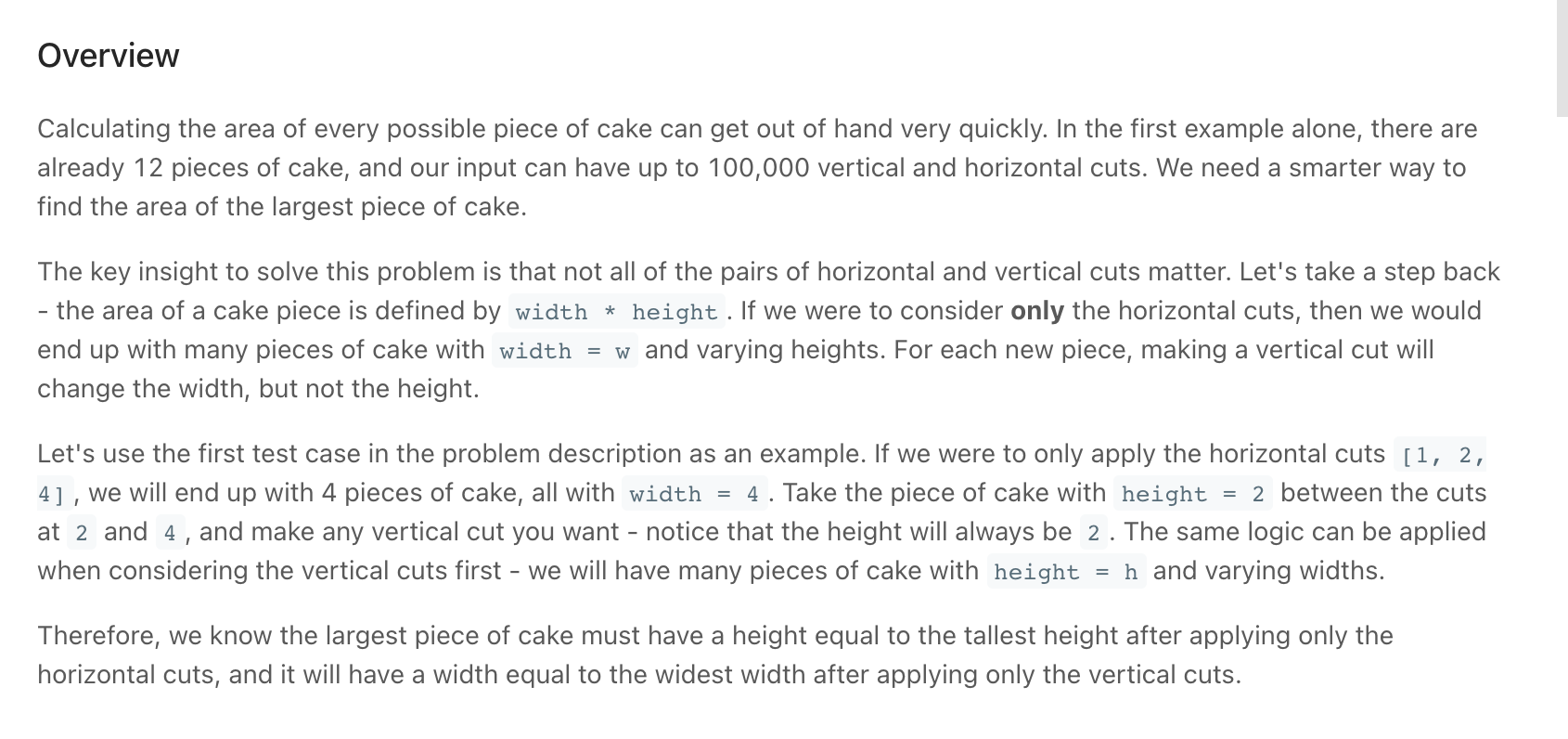Screen Recording 2021-10-19 at 08.14.12.mov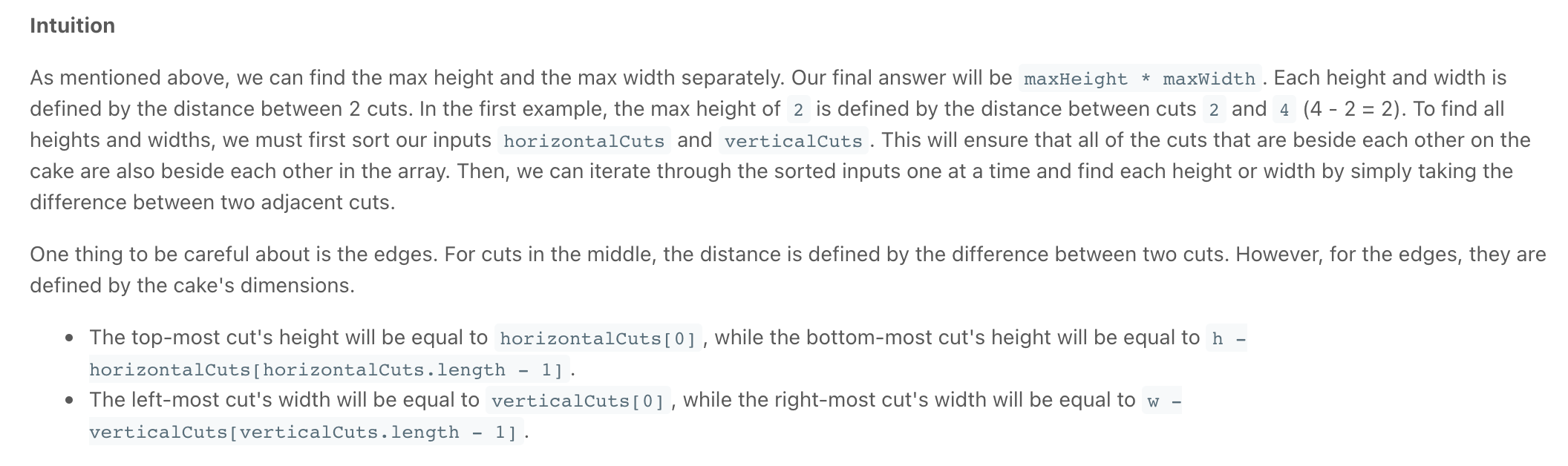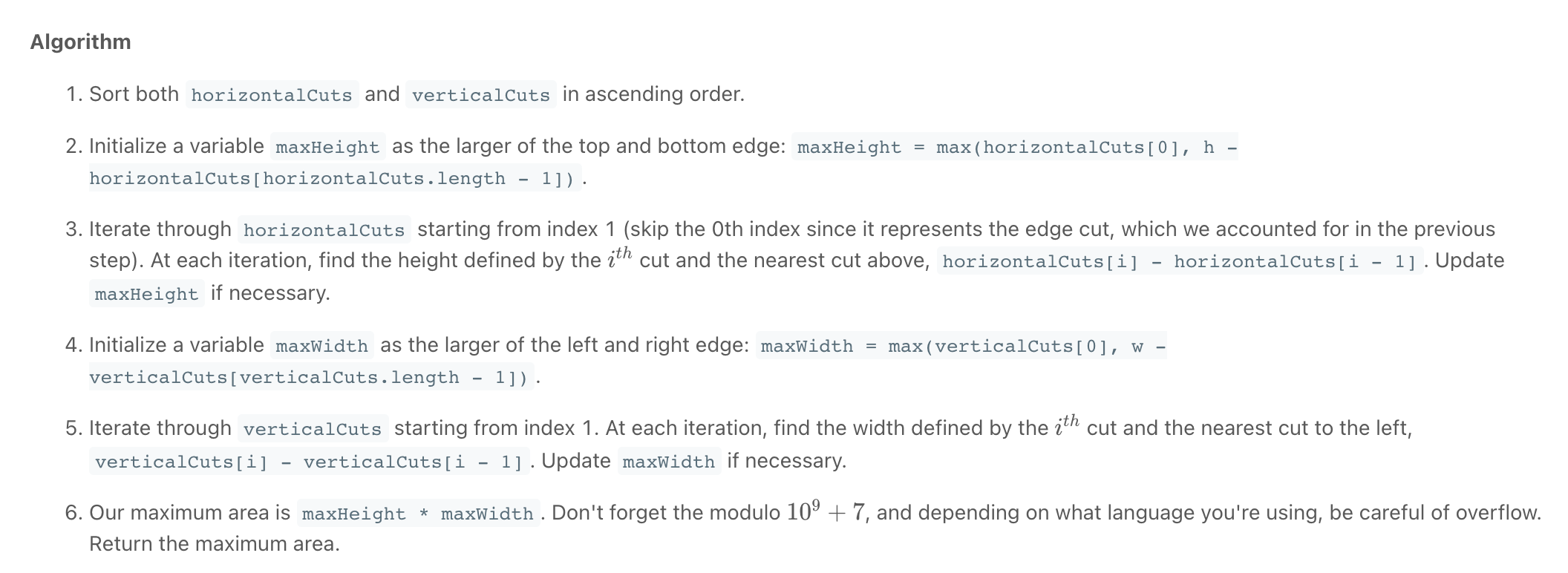"""
Maximum Area of a Piece of Cake After Horizontal and Vertical Cuts

You are given a rectangular cake of size h x w and two arrays of integers horizontalCuts and verticalCuts where:
horizontalCuts[i] is the distance from the top of the rectangular cake to the ith horizontal cut and similarly, and
verticalCuts[j] is the distance from the left of the rectangular cake to the jth vertical cut.
Return the maximum area of a piece of cake after you cut at each horizontal and vertical position provided in the arrays horizontalCuts and verticalCuts.
Since the answer can be a large number, return this modulo 109 + 7.

https://leetcode.com/problems/maximum-area-of-a-piece-of-cake-after-horizontal-and-vertical-cuts/
"""

class Solution:
def maxArea(self, h, w, horizontalCuts, verticalCuts):
"""
Note that: If we were to consider only the horizontal cuts, then we would end up with many pieces of cake with width = w and varying heights.
For each new piece, making a vertical cut will change the width, but not the height.
- when considering the heights, once we find the largest height, that piece is applicable to all the different possible widths
- when considering the widths, once we find the largest width, that piece is applicable to all heights

Therefore, we know the largest piece of cake must have a height equal to the tallest height after applying only the horizontal cuts,
and it will have a width equal to the widest width after applying only the vertical cuts.
"""

# Start by sorting the inputs
horizontalCuts.sort()
verticalCuts.sort()

max_height = max(horizontalCuts, h - horizontalCuts[-1])
for i in range(1, len(horizontalCuts)):
max_height = max(max_height,
horizontalCuts[i] - horizontalCuts[i - 1])

max_width = max(verticalCuts, w - verticalCuts[-1])
for i in range(1, len(verticalCuts)):
max_width = max(max_width, verticalCuts[i] - verticalCuts[i - 1])

return (max_height * max_width) % (10**9 + 7)

• Candy Crush

"""
Candy Crush:

This question is about implementing a basic elimination algorithm for Candy Crush:
Given a 2D integer array board representing the grid of candy, different positive integers board[i][j] represent different types of candies.
A value of board[i][j] = 0 represents that the cell at position (i, j) is empty.
The given board represents the state of the game following the player's move.

Prerequisite:

https://leetcode.com/problems/candy-crush/
"""

from typing import List

class Solution:

# 1. Check for vertical match
# 2. Check for horizontal match
# 3. Gravity
# 4. repeat till board is clean
# cells to be crushed will be marked as negative
def candyCrush(self, board: List[List[int]]):

h = len(board)
w = len(board)

toBeCrushed = False

#  1. Check for vertical match then
# negate the found (three cells)
# Note: (don't check the last two as they'll already have been checked)
for x in range(h-2):
for y in range(w):

# check a cell and the two below it then
# if they are equal, mark them (negate their values)
if abs(board[x][y]) == abs(board[x+1][y]) == abs(board[x+2][y]) != 0:
board[x][y] = board[x+1][y] = board[x+2][y] = - \
abs(board[x][y])
toBeCrushed = True

# 2. Check for horizontal match (similar to above)
for y in range(w-2):
for x in range(h):

# check a cell and the two to its right
# if they are equal, mark them
if abs(board[x][y]) == abs(board[x][y+1]) == abs(board[x][y+2]) != 0:
board[x][y] = board[x][y+1] = board[x][y+2] = - \
abs(board[x][y])
toBeCrushed = True

# 3. Gravity
# iterate through each column, then for each column,
# move positive values to the bottom then set the rest to 0.
if toBeCrushed:  # not needed but speeds it up

# # find negative values then, replace it with the ones above it using two pointers
# it's easier to iterate from the bottom
for y in range(w):

# will stick on negative values till they are replaced
anchor = h - 1  # starts off at the bottom

for x in reversed(range(h)):

# Replacing the negative values:
# check if positive, if positive,
#   we replace the last anchored cell[anchor][y] with the current cell([x],[y])
#   then we move the anchor up
# (skip this on -ve values, wait till we hit a +ve value to replace it)
if board[x][y] > 0:
# FYI, the first cells, if possitive will be replaced by themselves
board[anchor][y] = board[x][y]
anchor -= 1  # move anchor up

# deal with where the anchor last stuck,
# all the values of cells from the anchor upwards to be marked as 0
while anchor >= 0:
board[anchor][y] = 0
anchor -= 1

# Repeat till the board is clean
if toBeCrushed:
return self.candyCrush(board)
else:
return board

"""
Example:

Input:
board =
[[110,5,112,113,114],[210,211,5,213,214],[310,311,3,313,314],[410,411,412,5,414],[5,1,512,3,3],[610,4,1,613,614],[710,1,2,713,714],[810,1,2,1,1],[1,1,2,2,2],[4,1,4,4,1014]]

Output:
[[0,0,0,0,0],[0,0,0,0,0],[0,0,0,0,0],[110,0,0,0,114],[210,0,0,0,214],[310,0,0,113,314],[410,0,0,213,414],[610,211,112,313,614],[710,311,412,613,714],[810,411,512,713,1014]]
"""

"""
Full Question:

This question is about implementing a basic elimination algorithm for Candy Crush.

Given a 2D integer array board representing the grid of candy, different positive integers board[i][j] represent different types of candies. A value of board[i][j] = 0 represents that the cell at position (i, j) is empty. The given board represents the state of the game following the player's move. Now, you need to restore the board to a stable state by crushing candies according to the following rules:

If three or more candies of the same type are adjacent vertically or horizontally, "crush" them all at the same time - these positions become empty.
After crushing all candies simultaneously, if an empty space on the board has candies on top of itself, then these candies will drop until they hit a candy or bottom at the same time. (No new candies will drop outside the top boundary.)
After the above steps, there may exist more candies that can be crushed. If so, you need to repeat the above steps.
If there does not exist more candies that can be crushed (ie. the board is stable), then return the current board.
You need to perform the above rules until the board becomes stable, then return the current board.

Note:

The length of board will be in the range [3, 50].
The length of board[i] will be in the range [3, 50].
Each board[i][j] will initially start as an integer in the range [1, 2000].
"""

• Set Matrix Zeroes

"""
Set Matrix Zeroes:

Given an m x n matrix. If an element is 0, set its entire row and column to 0. Do it in-place.

A straight forward solution using O(mn) space is probably a bad idea.
A simple improvement uses O(m + n) space, but still not the best solution.
Could you devise a constant space solution?

Example 1:
Input: matrix =
[
[1,1,1],
[1,0,1],
[1,1,1]]
Output:
[
[1,0,1],
[0,0,0],
[1,0,1]
]
Example 2:
Input: matrix =
[
[0,1,2,0],
[3,4,5,2],
[1,3,1,5]
]
Output:
[
[0,0,0,0],
[0,4,5,0],
[0,3,1,0]
]

# Input: valid matrix
# Output: None
# Constraints:
- edit array in-place

https://leetcode.com/problems/set-matrix-zeroes/
"""

class Solution:
def setZeroes(self, matrix):
flag = "&"

# # Find Matrix Zeroes
first_col = first_row = False
for row in range(len(matrix)):
for col in range(len(matrix)):
if matrix[row][col] == 0:

# mark first row and col to be erased
matrix[row] = flag  # mark first col of row
matrix[col] = flag  # mark first row of col

if col == 0:
first_col = True
if row == 0:
first_row = True

# # Set Matrix Zeroes
# # do not set matrix zeros for the first row & column as they are used as guides for marking
# deal with rows
for row in range(1, len(matrix)):
if matrix[row] == flag:
for col in range(len(matrix)):
matrix[row][col] = 0
# deal with cols
for col in range(1, len(matrix)):
if matrix[col] == flag:
for row in range(len(matrix)):
matrix[row][col] = 0

# # Set Matrix Zeroes for the first row & col
if first_col:
for i in range(len(matrix)):
matrix[i] = 0
if first_row:
for i in range(len(matrix)):
matrix[i] = 0

return matrix

• Spiral Matrix

"""
Spiral Matrix:

Given a matrix of m x n elements (m rows, n columns), return all elements of the matrix in spiral order.
Given an m x n matrix, return all elements of the matrix in spiral order.
Example 1:
Input: matrix = [[1,2,3],[4,5,6],[7,8,9]]
Output: [1,2,3,6,9,8,7,4,5]
Example 2:
Input: matrix = [[1,2,3,4],[5,6,7,8],[9,10,11,12]]
Output: [1,2,3,4,8,12,11,10,9,5,6,7]

https://leetcode.com/problems/spiral-matrix/
"""

# O(n) time | 0(n) space
def spiralTraverse1(array):
result = []

rowBegin = columnBegin = 0
rowEnd = len(array) - 1
columnEnd = len(array) - 1

isTop = True
isRight = isLeft = isBottom = False

while (rowBegin <= rowEnd and (isBottom or isTop)) or \
(columnBegin <= columnEnd and (isRight or isLeft)):
# the and (isRight or isLeft) helps prevent addition of an extra element after the traversal is complete
# print(result)
if isTop:
for col in range(columnBegin, columnEnd + 1):
result.append(array[rowBegin][col])
rowBegin += 1
isTop = False
isRight = True
elif isRight:
for row in range(rowBegin, rowEnd+1):
result.append(array[row][columnEnd])
columnEnd -= 1
isBottom = True
isRight = False
elif isBottom:
for col in reversed(range(columnBegin, columnEnd+1)):
result.append(array[rowEnd][col])
rowEnd -= 1
isLeft = True
isBottom = False
elif isLeft:
for row in reversed(range(rowBegin, rowEnd + 1)):
result.append(array[row][columnBegin])
columnBegin += 1
isTop = True
isLeft = False
return result

def spiralTraverse(array):
output = []

left = 0
right = len(array) - 1
top = 0
bottom = len(array) - 1
while left <= right or top <= bottom:

# top
if top <= bottom:
for idx in range(left, right+1):
output.append(array[top][idx])
top += 1

# right
if left <= right:
for idx in range(top, bottom+1):
output.append(array[idx][right])
right -= 1

# bottom
if top <= bottom:
for idx in reversed(range(left, right+1)):
output.append(array[bottom][idx])
bottom -= 1

# left
if left <= right:
for idx in reversed(range(top, bottom+1)):
output.append(array[idx][left])
left += 1

return output

# O(n) time | 0(n) space
def spiralTraverse0(array):
result = []

top = left = 0
bottom = len(array) - 1
right = len(array) - 1

while (top <= bottom) and (left <= right):

# top
for col in range(left, right + 1):
result.append(array[top][col])

# right
for row in range(top + 1, bottom+1):
result.append(array[row][right])

# bottom
for col in reversed(range(left, right)):
# Handle the edge case when there's a single row
# in the middle of the matrix. In this case, we don't
# want to double-count the values in this row, which
# we've already counted in the first for loop above.
if top == bottom:
break
result.append(array[bottom][col])

# left
for row in reversed(range(top + 1, bottom)):
# Handle the edge case when there's a single column
# in the middle of the matrix. In this case, we don't
# want to double-count the values in this column, which
# we've already counted in the second for loop above.
if left == right:
break
result.append(array[row][left])

top += 1
bottom -= 1
left += 1
right -= 1

return result


Tips:

• Array problems often have simple brute-force solutions that use O(n) space, but there are subtler solutions that use the array itself to reduce space complexity to O(1).
• Instead of deleting an entry (which requires moving all entries to its right), consider overwriting it.
• Be comfortable with writing code that operates on subarrays.
• It’s incredibly easy to make off-by-1 errors when operating on arrays—reading past the last element of an array is a common error that has catastrophic consequences.
• Don’t worry about preserving the integrity of the array (sortedness, keeping equal entries together, etc.) until it is time to return.
• An array can serve as a good data structure when you know the distribution of the elements in advance. For example, a Boolean array of length W is a good choice for representing a subset of {0, 1, . . . , W − 1}. (When using a Boolean array to represent a subset of {1, 2, 3, . . . , n}, allocate an array of size n + 1 to simplify indexing.) .
• When operating on 2D arrays, use parallel logic for rows and for columns.
• Sometimes it’s easier to simulate the specification than to analytically solve for the result. For example, rather than writing a formula for the i-th entry in the spiral order for an n × n matrix, just compute the output from the beginning.
• The basic operations are: len(A), A.append(42), A.remove(2), and A.insert(3, 28), A.reverse() (in-place), reversed(A) (returns an iterator), A.sort() (in-place), sorted(A) (returns a copy), del A[i] (deletes the i-th element), and del A[i:j] (removes the slice).
• Understand how copy works, i.e., the difference between B = A and B = list(A). Understand what a deep copy is, and how it differs from a shallow copy, i.e., how copy.copy(A) differs from copy.deepcopy(A).

Functions

.append(item) adds an item to the end of the list.

.insert(index, item) adds an item at the given index in the list.

.remove(item) removes an item from the list.

.pop(index) removes the item at the given index.

.count(item) returns a count of how many times an item occurs in the list.

.reverse() reverses items in the list in place reversed(list) (returns an iterator).

.sort() sorts the list in place. By default, the list is sorted ascending. You can specify sort(reverse=True), to sort descending. sorted(list) (returns a copy)

max(list) returns the maximum value.

min(list) returns the minimum value.

del list[i] (deletes the i-th element), and del list[i:j] (removes the slice).

Find index [1,2,3,4].index(3)) → 2 *

Untitled

# Python list methods
my_list = [3, 8, 1, 6, 0, 8, 4]

# Output: 1
print(my_list.index(8))

# Output: 2
print(my_list.count(8))

my_list.sort()

# Output: [0, 1, 3, 4, 6, 8, 8]
print(my_list)

my_list.reverse()

# Output: [8, 8, 6, 4, 3, 1, 0]
print(my_list)

# Deleting list items
my_list = ['p', 'r', 'o', 'b', 'l', 'e', 'm']

# delete one item
del my_list

print(my_list) # ['p', 'r', 'b', 'l', 'e', 'm']

# delete multiple items
del my_list[1:5]

print(my_list) # ['p', 'm']

# delete entire list
del my_list

print(my_list) # Error: List not defined

my_list = ['p','r','o','b','l','e','m']
my_list.remove('p')

# Output: ['r', 'o', 'b', 'l', 'e', 'm', 'p']
print(my_list)

# Demonstration of list insert() method
odd = [1, 9]
odd.insert(1,3)

print(odd) # [1, 3, 9]

odd[2:2] = [5, 7]

print(odd) # [1, 3, 5, 7, 9]


### List comprehensions

cubes = [i**3 for i in range(5)] # [0, 1, 8, 27, 64]
print([i*2 for i in range(5)]) # [0, 2, 4, 6, 8]

evens=[i**2 for i in range(10) if i**2 % 2 == 0] # [0, 4, 16, 36, 64]


Slicing

list[::2] returns every other element from the list (indices 0, 2, .. )

# substitute
word = "0123456789"
temp_w = list(word)
temp_w[2:4] = "t"
print(temp_w)


## Tuple

Python Tuple

Unlike lists, tuples are immutable.

# Changing tuple values
my_tuple = (4, 2, 3, [6, 5])

# TypeError: 'tuple' object does not support item assignment
# my_tuple = 9

# However, item of mutable element can be changed
my_tuple = 9    # Output: (4, 2, 3, [9, 5])
print(my_tuple)

# Tuples can be reassigned
my_tuple = ('p', 'r', 'o', 'g', 'r', 'a', 'm', 'i', 'z')

# Output: ('p', 'r', 'o', 'g', 'r', 'a', 'm', 'i', 'z')
print(my_tuple)


## Pro tips

### Multiplying lists can just create several copies to the same list

Python list multiplication: [[...]]*3 makes 3 lists which mirror each other when modified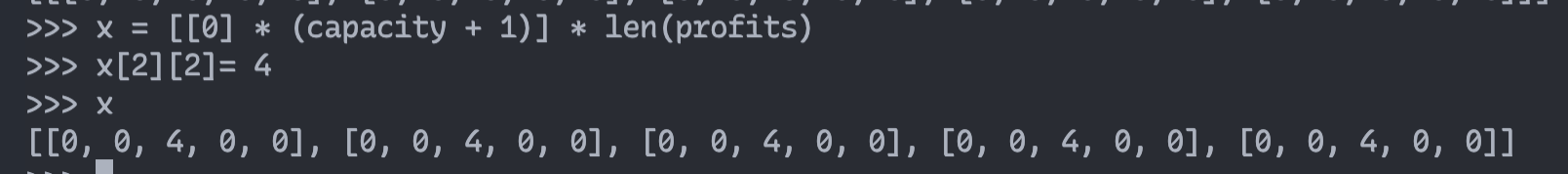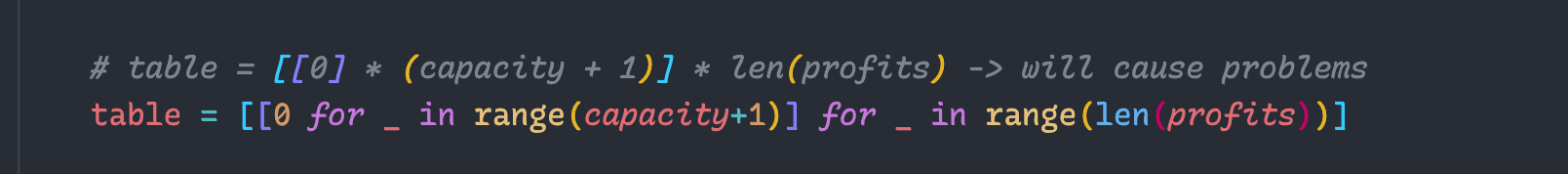# Sets

### Examples

Hare & Tortoise Algorithm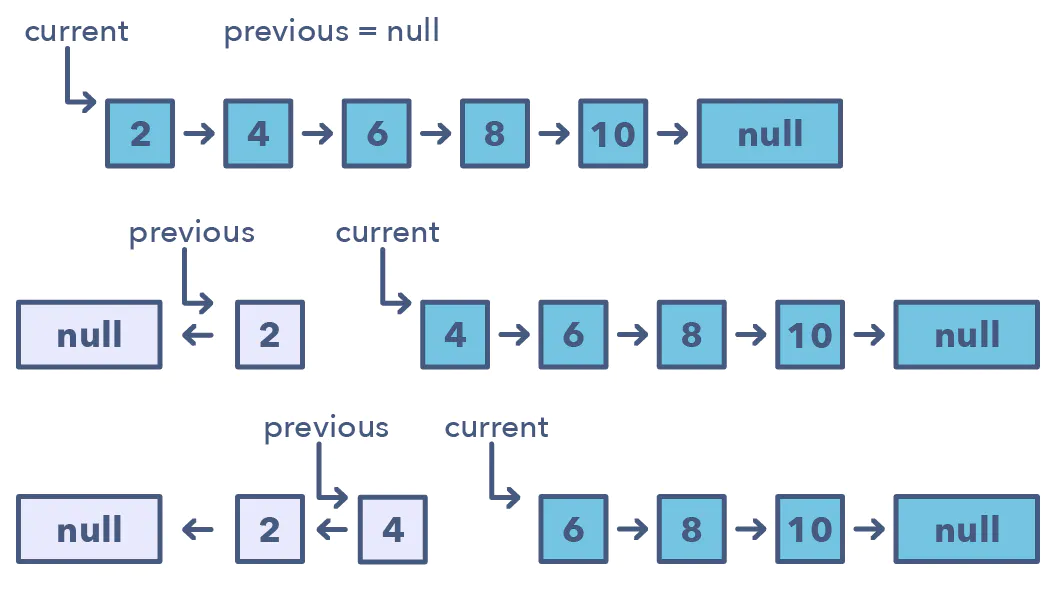"""

"""

class ListNode:
def __init__(self, val=0, next=None):
self.val = val
self.next = next

# O(n) time | O(1) space
class Solution:

prev = None

while current is not None:
# store next because we will loose track of it
nxt = current.next

# reverse pointer (point backwords)
current.next = prev

# # move on to next node
# in the next iteration, the current current will be the prev
prev = current
# in the next iteration, the current current.next will be the current
current = nxt

return prev

prev = None
while curr is not None:
nxt = curr.next
curr.next = prev
prev = curr
curr = nxt
return prev


"""

Given the head of a singly linked list and two integers left and right where left <= right,
reverse the nodes of the list from position left to position right, and return the reversed list.
Follow up: Could you do it in one pass?
Example 1:
Input: head = [1,2,3,4,5], left = 2, right = 4
Output: [1,4,3,2,5]
Example 2:
Input: head = , left = 1, right = 1
Output: 
"""

class Solution:
def reverseBetween(self, head: ListNode, left: int, right: int):
if left == right:

before_sublist = after_sublist = None
sublist_start = sublist_end = None

prev = None
count = 1
while curr:
if count == left-1:
before_sublist = curr
elif count == left:
sublist_start = curr
elif count == right:
sublist_end = curr
elif count == right+1:
after_sublist = curr

# reverse sublist
nxt = curr.next
if count > left and count <= right:
curr.next = prev

prev = curr
curr = nxt
count += 1

# correct start and end of sublist
if before_sublist is None:
sublist_start.next = after_sublist
else:
before_sublist.next = sublist_end
sublist_start.next = after_sublist

"""

"""

class Solution_:
def reverseBetween(self, head: ListNode, left: int, right: int):
prev = None

# # find where reversing begins
for _ in range(left-1):  # the 1st node is at pos 1
prev = curr
curr = curr.next

# we cannot use before_reverse.next when left is at 1, coz there is no before_reverse so we use start_reverse
# store the last non reversed(not to be reversed) node
start_reverse = curr
# will be the tail of the last reversed list
before_reverse = prev

# # reverse a section
for _ in range(left, right+1):
nxt = curr.next
curr.next = prev
prev = curr
curr = nxt

# # merge the reversed section (fix the reversed list position in the larger list)
# the (left - 1) node to point to right
if before_reverse and before_reverse.next:
# before_reverse.next = last reversed node (prev)
before_reverse.next = prev

else:
# if we started reversing from 1, then the last item reversed will be put at 1 (head)

# the first reversed (left) node to point to the node at (right + 1)
start_reverse.next = curr


• Reorder List *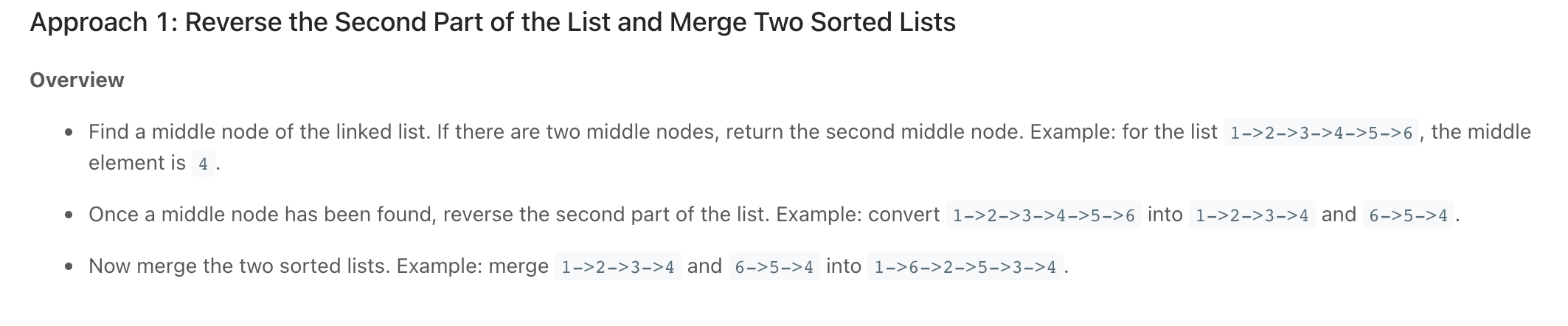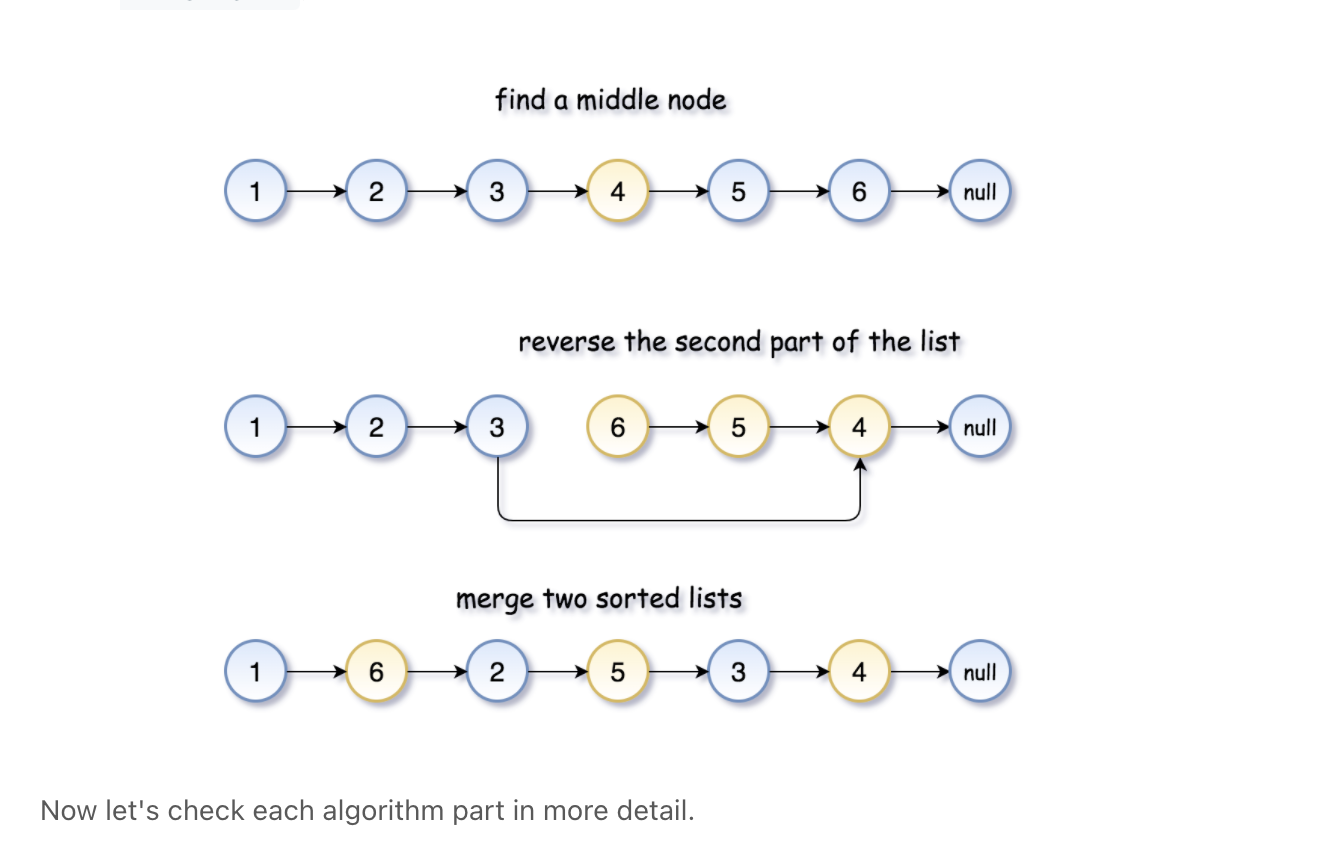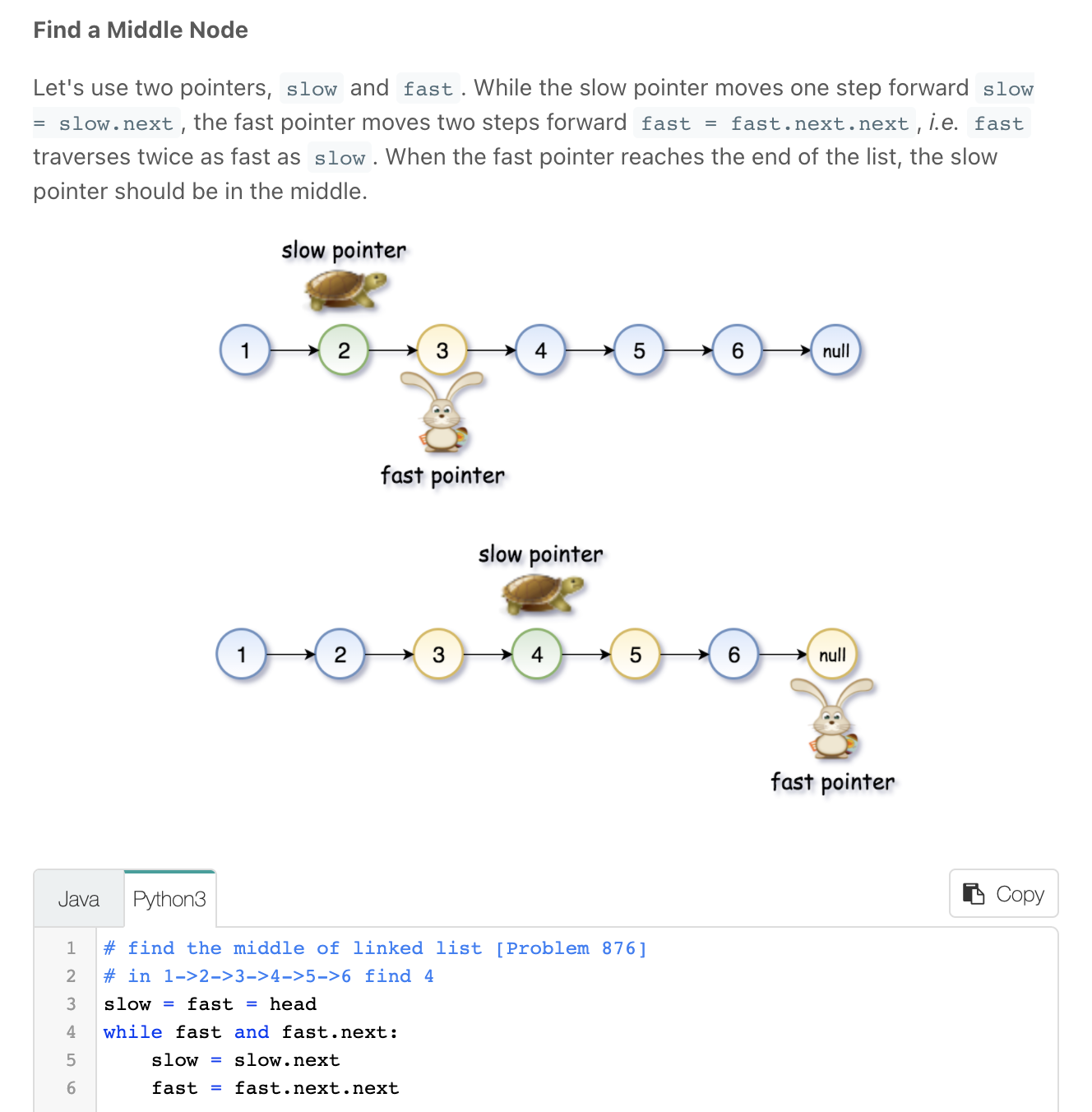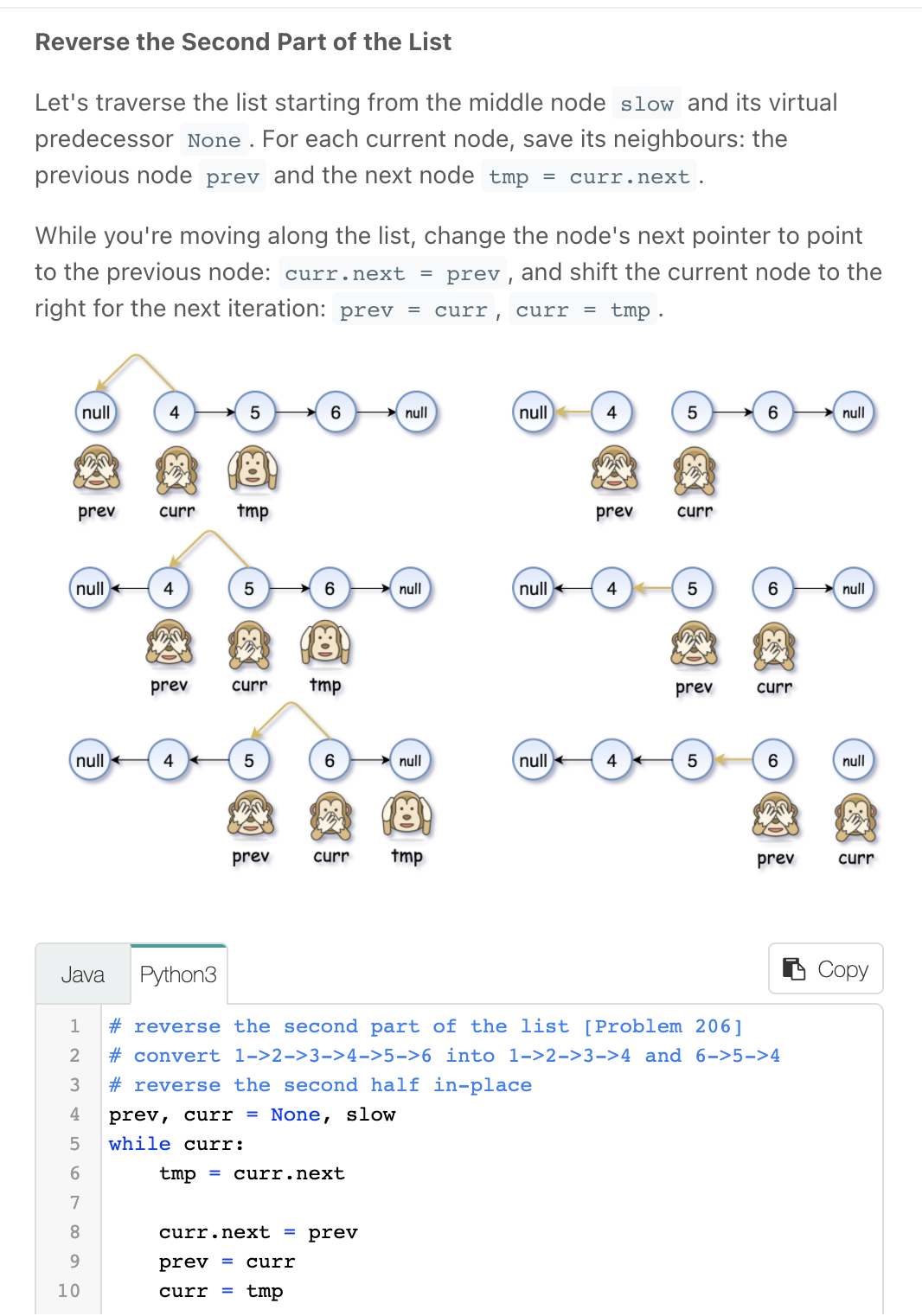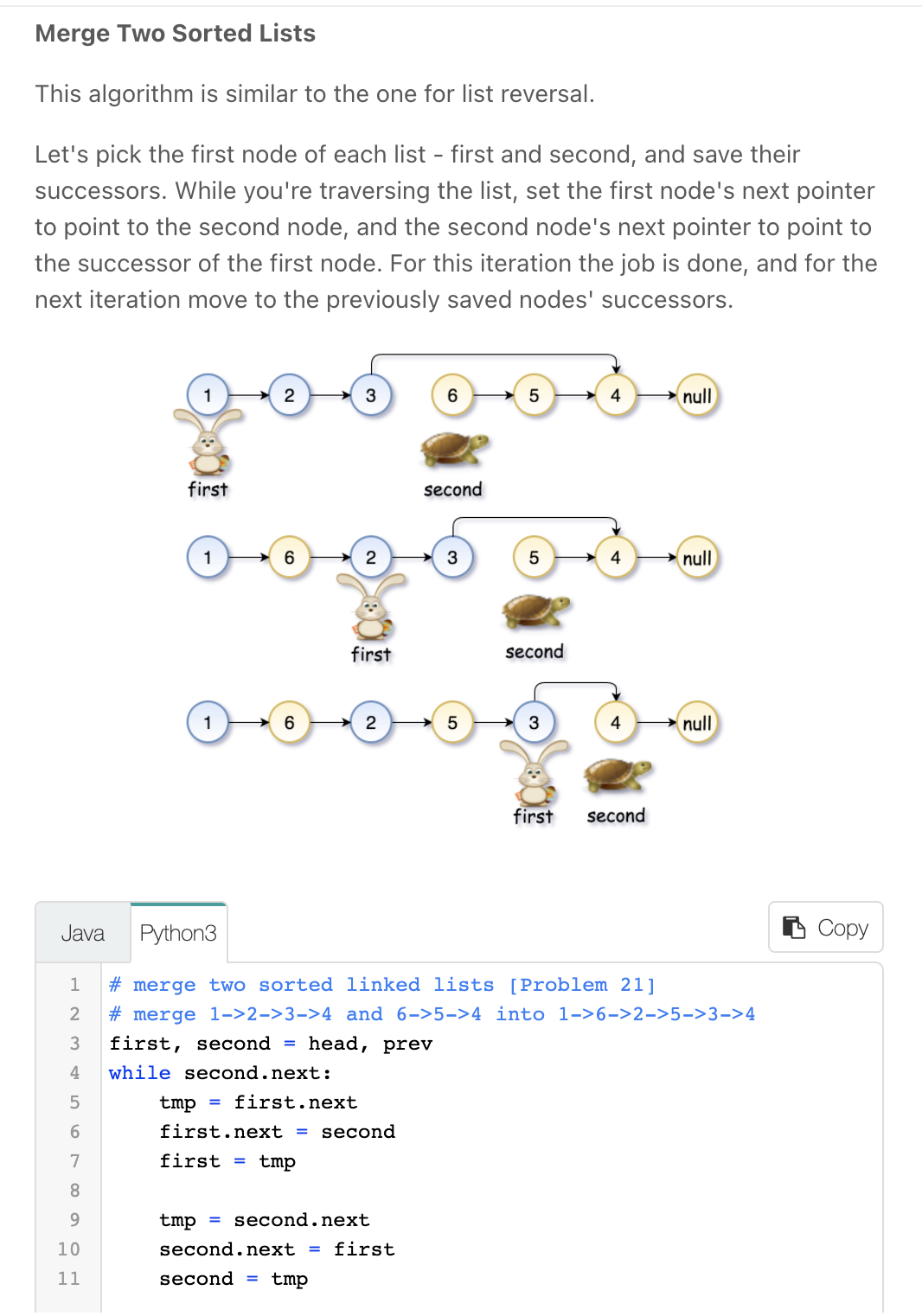"""
Reorder List

You are given the head of a singly linked-list. The list can be represented as:
L0 → L1 → … → Ln - 1 → Ln
Reorder the list to be on the following form:
L0 → Ln → L1 → Ln - 1 → L2 → Ln - 2 → …
You may not modify the values in the list's nodes. Only nodes themselves may be changed.

Example 1:
Output: [1,4,2,3]
Example 2:
Output: [1,5,2,4,3]

https://leetcode.com/problems/reorder-list
"""

"""
This problem is a combination of these three easy problems:
Merge Two Sorted Lists.
"""

class Solution:
return

# find the middle of linked list [Problem 876]
# in 1->2->3->4->5->6 find 4
while fast and fast.next:
slow = slow.next
fast = fast.next.next

# reverse the second part of the list [Problem 206]
# convert 1->2->3->4->5->6 into 1->2->3->4 and 6->5->4
# reverse the second half in-place
prev, curr = None, slow
while curr:
curr.next, prev, curr = prev, curr, curr.next

# merge two sorted linked lists [Problem 21]
# merge 1->2->3->4 and 6->5->4 into 1->6->2->5->3->4
while second.next:
first.next, first = second, first.next
second.next, second = first, second.next

• Find the start of linked list cycle

def detectCycle(self, head):

# # find cycle
while True:
if fast is None or fast.next is None:  # find invalid
return None

slow = slow.next
fast = fast.next.next
if slow == fast:
break

# # find start of cycle
# the (dist) head to the start of the cycle ==
#   the (dist) meeting point to the start of the cycle
two = fast
while one != two:
one = one.next
two = two.next
return one

• Check/Store unique nodes (not necessarily with unique values)

Not necessarily with unique values → meaning we can have two different nodes with the same value

• Merge K Sorted Lists

"""

Given the head of a linked list, rotate the list to the right by k places.

Example 1:
Input: head = [1,2,3,4,5], k = 2
Output: [4,5,1,2,3]
Example 2:
Input: head = [0,1,2], k = 4
Output: [2,0,1]

Constraints:
The number of nodes in the list is in the range [0, 500].
-100 <= Node.val <= 100
0 <= k <= 2 * 109

https://leetcode.com/problems/rotate-list/
"""

# class ListNode:
#     def __init__(self, val=0, next=None):
#         self.val = val
#         self.next = next

"""
- find length of list
- find end of list
- k %= length
- we'll have to pluck of the list from position length - k to the end
and place it a the beginning of the list
- get to node (length - k):
- hold k in a pointer (new_head)
- node (length - k) to point to null
- end to point to head

0 -> 1 -> 2 -> 3 -> 4 -> 5

2
4 -> 5 -> 0 -> 1 -> 2 -> 3

5
1 -> 2 -> 3 -> 4 -> 5 -> 0

"""

class Solution:
return

# # get tail and length
tail = None
length = 0
while curr:
tail = curr
curr = curr.next
length += 1

# # validate k
k %= length
if k == 0:

# # find new ending (length - k)
for _ in range(length - k - 1):
new_tail = new_tail.next

# # rotate
new_tail.next = None


• Merge Two Sorted Lists/Merge Linked Lists *

"""
Merge Two Sorted Lists/Merge Linked Lists:

Merge two sorted linked lists and return it as a sorted list.
The list should be made by splicing together the nodes of the first two lists.

https://leetcode.com/problems/merge-two-sorted-lists/
"""

"""
Have two pointers, one at l1 and another at l2
we will be merging l1 into l2
compare which one is smaller, add the appropriate and move the pointers forward and move the
"""

class ListNode:
def __init__(self, val=0, next=None):
self.val = val
self.next = next

class Solution:
def mergeTwoLists(self, l1: ListNode, l2: ListNode):
if l1 is None and l2 is None:
return None
elif l1 is None:
return l2
elif l2 is None:
return l1

one = l1
two = l2

if one.val < two.val:
temp_one = one
one = two
two = temp_one

prev_two = None
while one is not None and two is not None:
if one.val < two.val:
nxt = one.next
self.insertBetween(prev_two, two, one)
prev_two = one
one = nxt
else:
prev_two = two
two = two.next

while one is not None:  # add remaining one into two
prev_two.next = one
prev_two = prev_two.next
one = one.next

def insertBetween(self, left, right, new):
left.next = new
new.next = right

• Insert into a Sorted Circular Linked List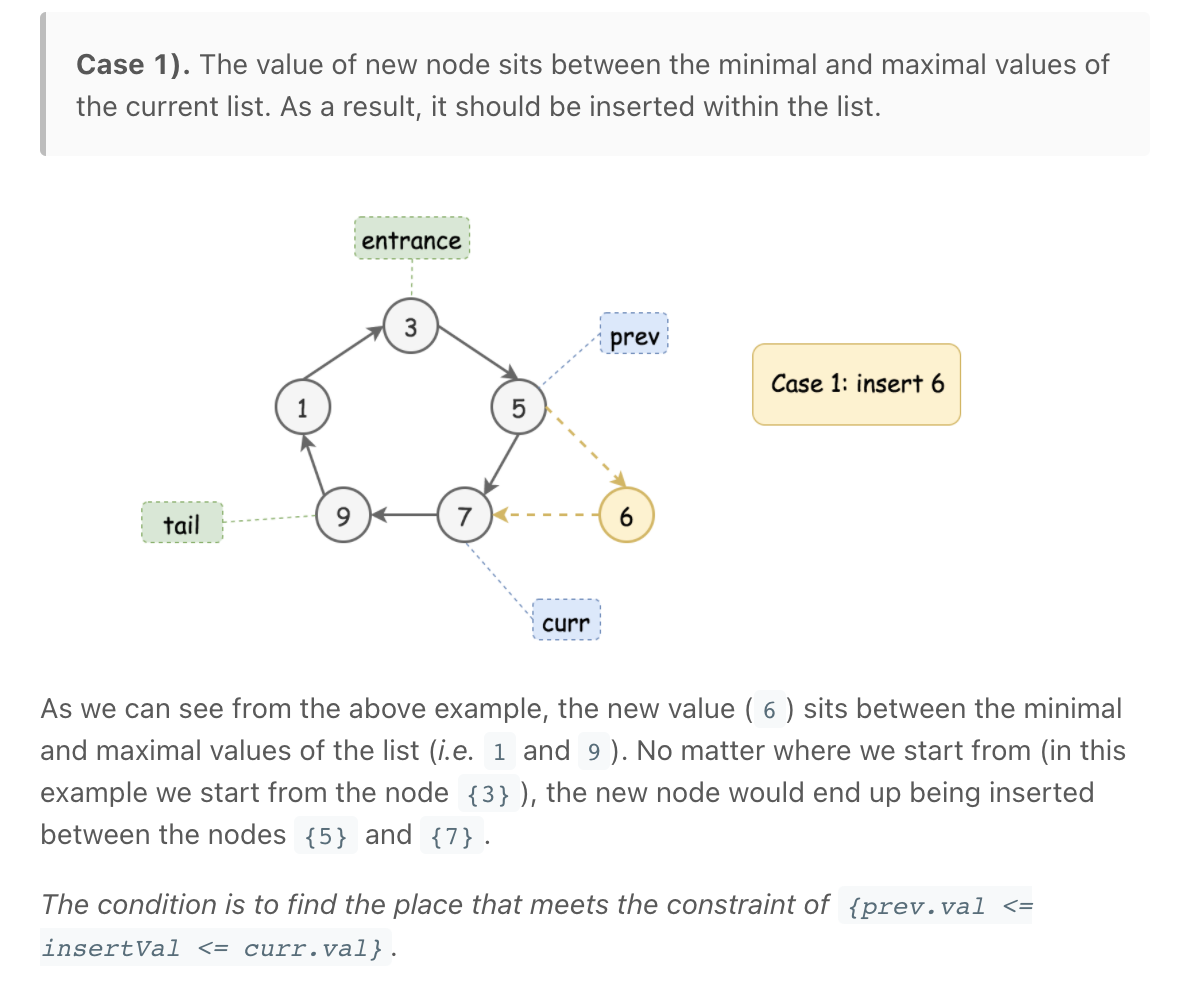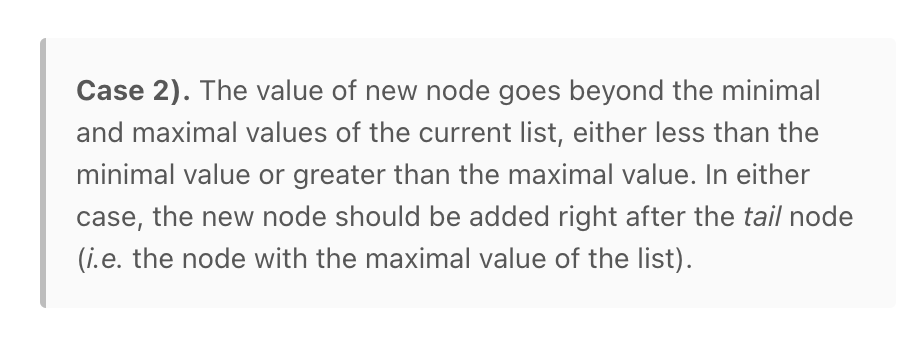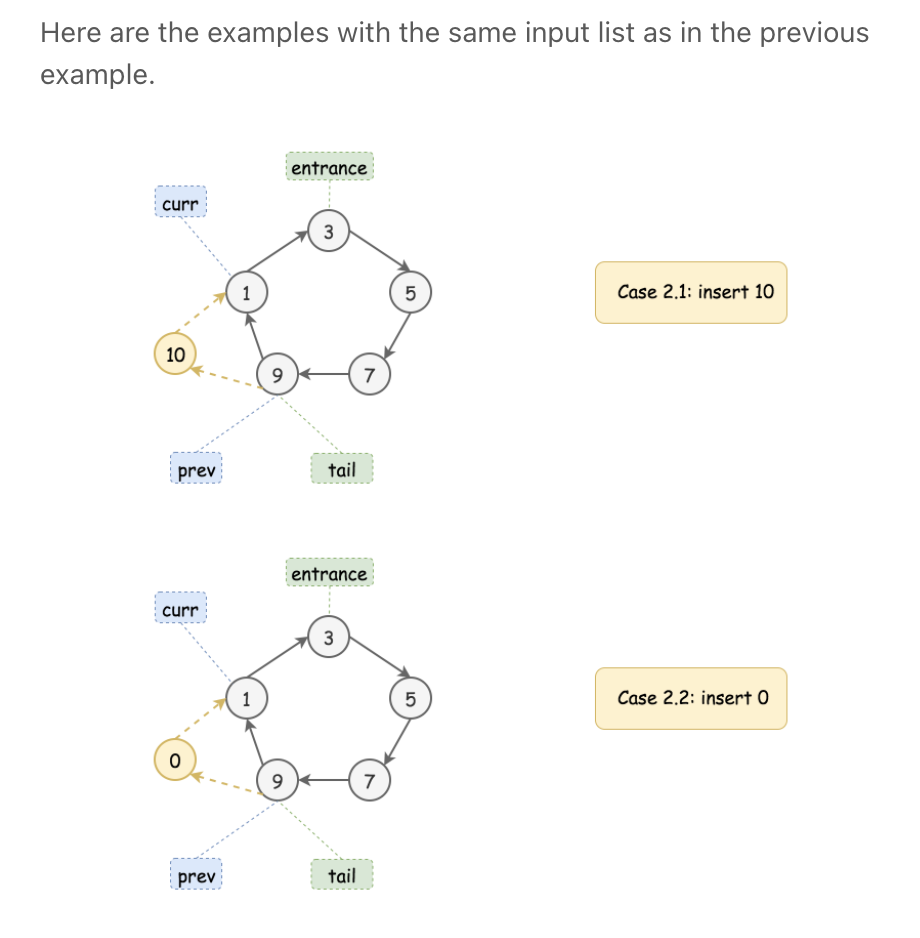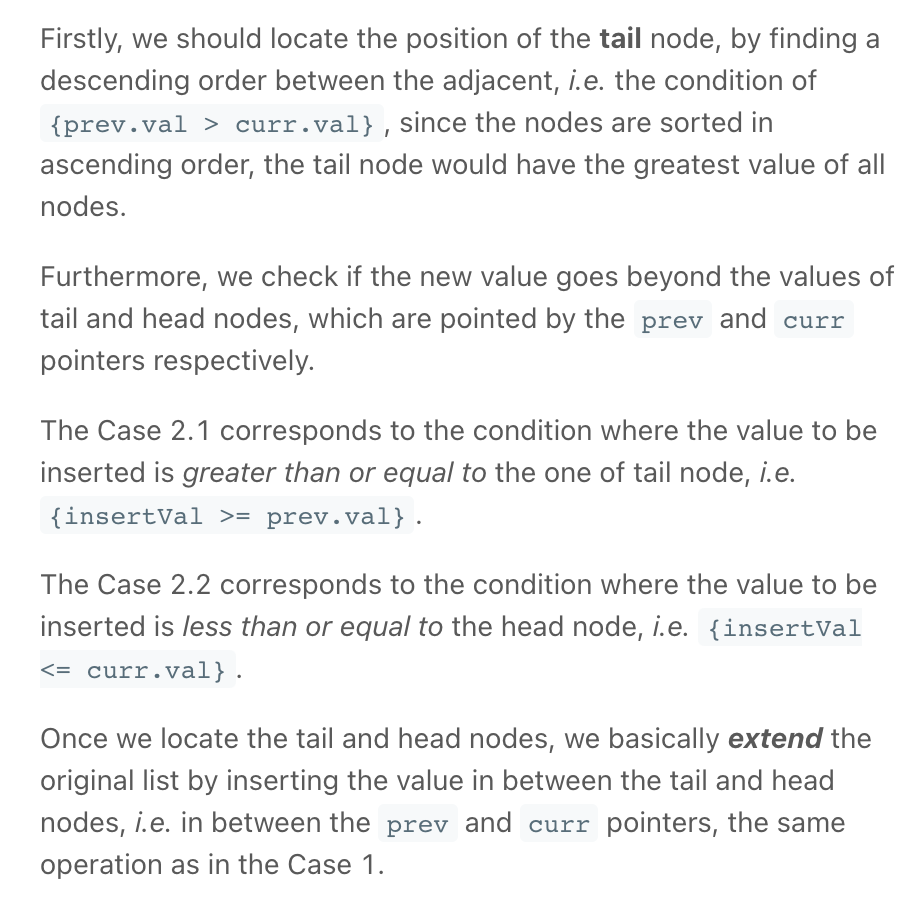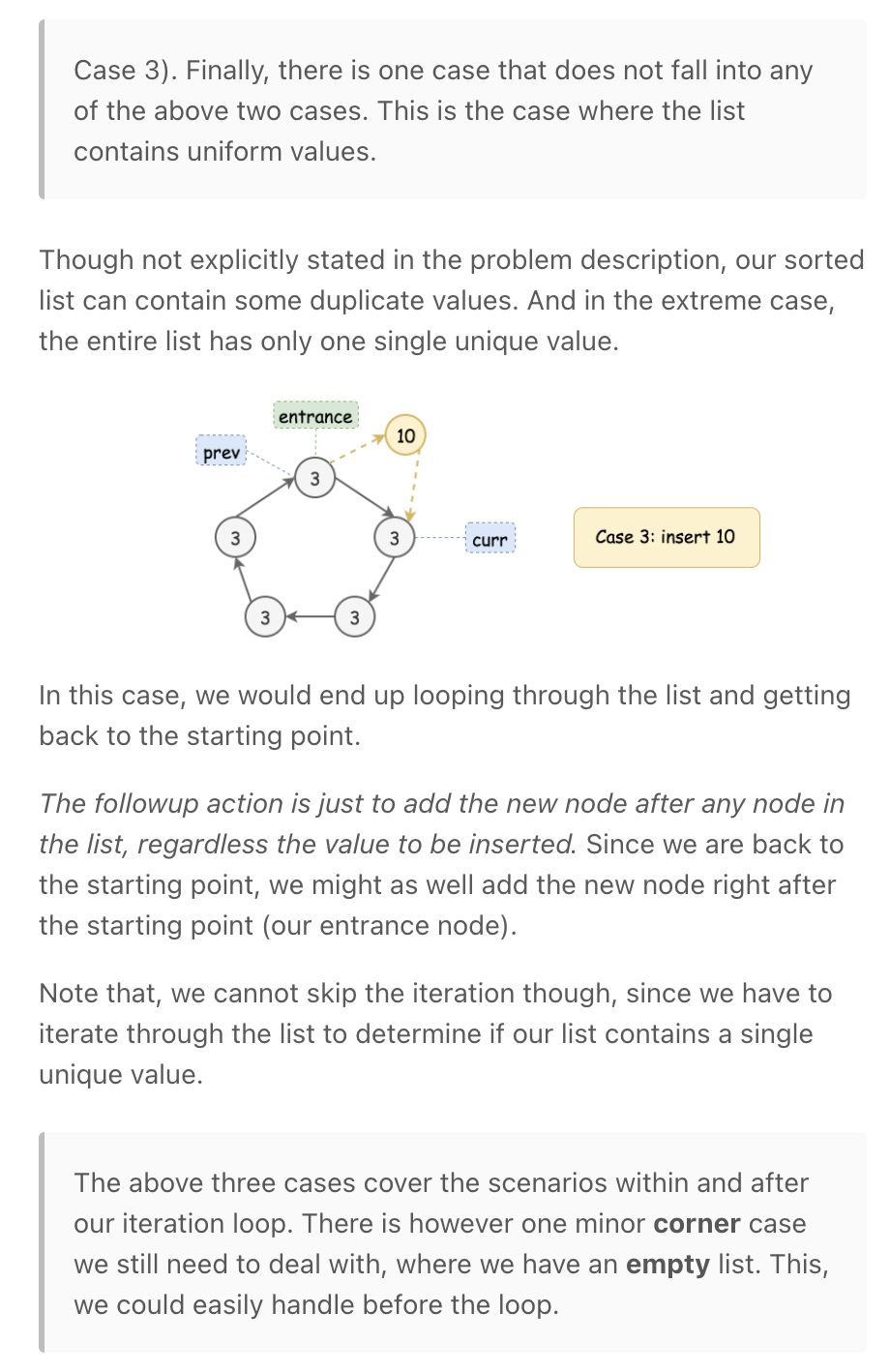"""
Insert into a Sorted Circular Linked List:

Given a Circular Linked List node, which is sorted in ascending order, write a function to insert a value insertVal into the list such that it remains a sorted circular list.
The given node can be a reference to any single node in the list and may not necessarily be the smallest value in the circular list.
If there are multiple suitable places for insertion, you may choose any place to insert the new value. After the insertion, the circular list should remain sorted.
If the list is empty (i.e., the given node is null), you should create a new single circular list and return the reference to that single node. Otherwise, you should return the originally given node.

Example 1:
Input: head = [3,4,1], insertVal = 2
Output: [3,4,1,2]
Explanation: In the figure above, there is a sorted circular list of three elements.
You are given a reference to the node with value 3, and we need to insert 2 into the list.
The new node should be inserted between node 1 and node 3. After the insertion, the list should look like this, and we should still return node 3.
Example 2:
Input: head = [], insertVal = 1
Output: 
Explanation: The list is empty (given head is null). We create a new single circular list and return the reference to that single node.
Example 3:
Input: head = , insertVal = 0
Output: [1,0]

"""

# Definition for a Node.
class Node:
def __init__(self, val=None, next=None):
self.val = val
self.next = next

class Solution:
def insert(self, head: 'Node', insertVal: int):
node = Node(insertVal)

# empty list
node.next = node
return node

# the or equal is to ensure the largest is the last node in such [3,3,3]
if curr.val < smallest.val:
smallest = curr
if curr.val >= largest.val:
largest = curr
curr = curr.next

# only one node or all nodes have a similar value
if smallest.val == largest.val:
largest.next = node
node.next = smallest

# is the largest or smallest value
elif insertVal <= smallest.val or insertVal >= largest.val:
largest.next = node
node.next = smallest

else:
prev = None
curr = None
while curr != smallest:
if curr is None:
curr = smallest
prev = largest

if insertVal < curr.val:
prev.next = node
node.next = curr
break

prev = curr
curr = curr.next


• Test for overlapping lists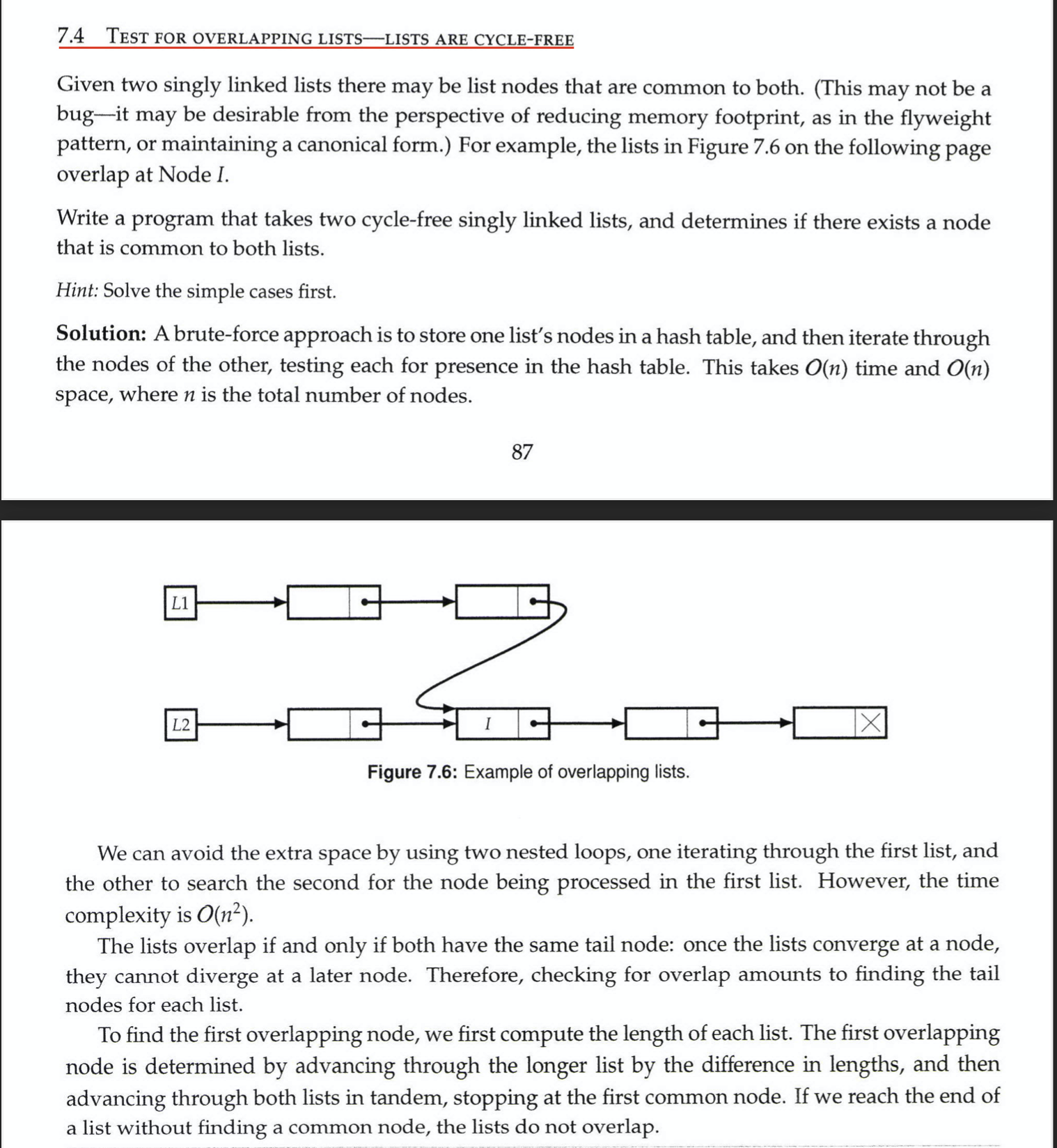• Remove Kth Node From End

"""
Remove Kth Node From End:

Write a function that takes in the head of a Singly Linked List and an integer k and removes the kth node from the end of the list.
The removal should be done in place, meaning that the original data structure should be mutated (no new structure should be created).
even if the head is the node that's supposed to be removed.
In other words, if the head is the node that's supposed to be removed, your function should simply mutate its value and next pointer.
Note that your function doesn't need to return anything.
You can assume that the input Linked List will always have at least two nodes and, more specifically, at least k nodes.
Each LinkedList node has an integer value as well as a next node pointing to the next node in the list or to None / null if it's the tail of the list.
https://www.algoexpert.io/questions/Remove%20Kth%20Node%20From%20End
"""

def __init__(self, value):
self.value = value
self.next = None

# O(n) time | O(n) space

# find node positions
positions = {}
count = 1
while curr is not None:
positions[count] = curr
curr = curr.next
count += 1

if k == 1:  # if node is the tail:
before_to_delete = positions[(count-(k))-1]
before_to_delete.next = None
else:
after_to_delete = positions[(count-(k))+1]
to_delete = positions[count-(k)]
to_delete.value = after_to_delete.value
to_delete.next = after_to_delete.next

# O(n) time | O(1) space

prev_left = None

counter = 1
# create length k space between right and left
while counter <= k:
right = right.next
counter += 1

# find end (move right past end)
while right is not None:
prev_left = left
left = left.next
right = right.next

# delete
if left.next is None:  # tail
prev_left.next = None
else:
nxt = left.next
left.value = nxt.value
left.next = nxt.next

before_left = None

counter = 1
while counter <= k:
right = right.next
counter += 1

while right is not None:
right = right.next
before_left = left
left = left.next

if before_left is None:  # remove head
left.value = left.next.value
left.next = left.next.next
else:
before_left.next = before_left.next.next

counter = 1
while counter <= k:  # not counter < k to ensure we say on the node_at_k.prev
right = right.next
counter += 1

if right is None:
left.value = left.next.value
left.next = left.next.next

else:
while right.next is not None:
left = left.next
right = right.next

left.next = left.next.next

"""
Remove Nth Node From End of List:

Given the head of a linked list, remove the nth node from the end of the list and return its head.
Follow up: Could you do this in one pass?
https://leetcode.com/problems/remove-nth-node-from-end-of-list/
"""

class ListNode:
def __init__(self, val=0, next=None):
self.val = val
self.next = next

class Solution:
def removeNthFromEnd(self, head: ListNode, n: int):

# keep distance
count = 1
while count != n:
count += 1
right = right.next

# find end
prev_left = None
while right is not None:
prev_left = left
left = left.next
right = right.next

# remove
if count == 1 and prev_left is None and right is None:  # list has single element
return None
elif prev_left is None:  # remove head

prev_left.next = left.next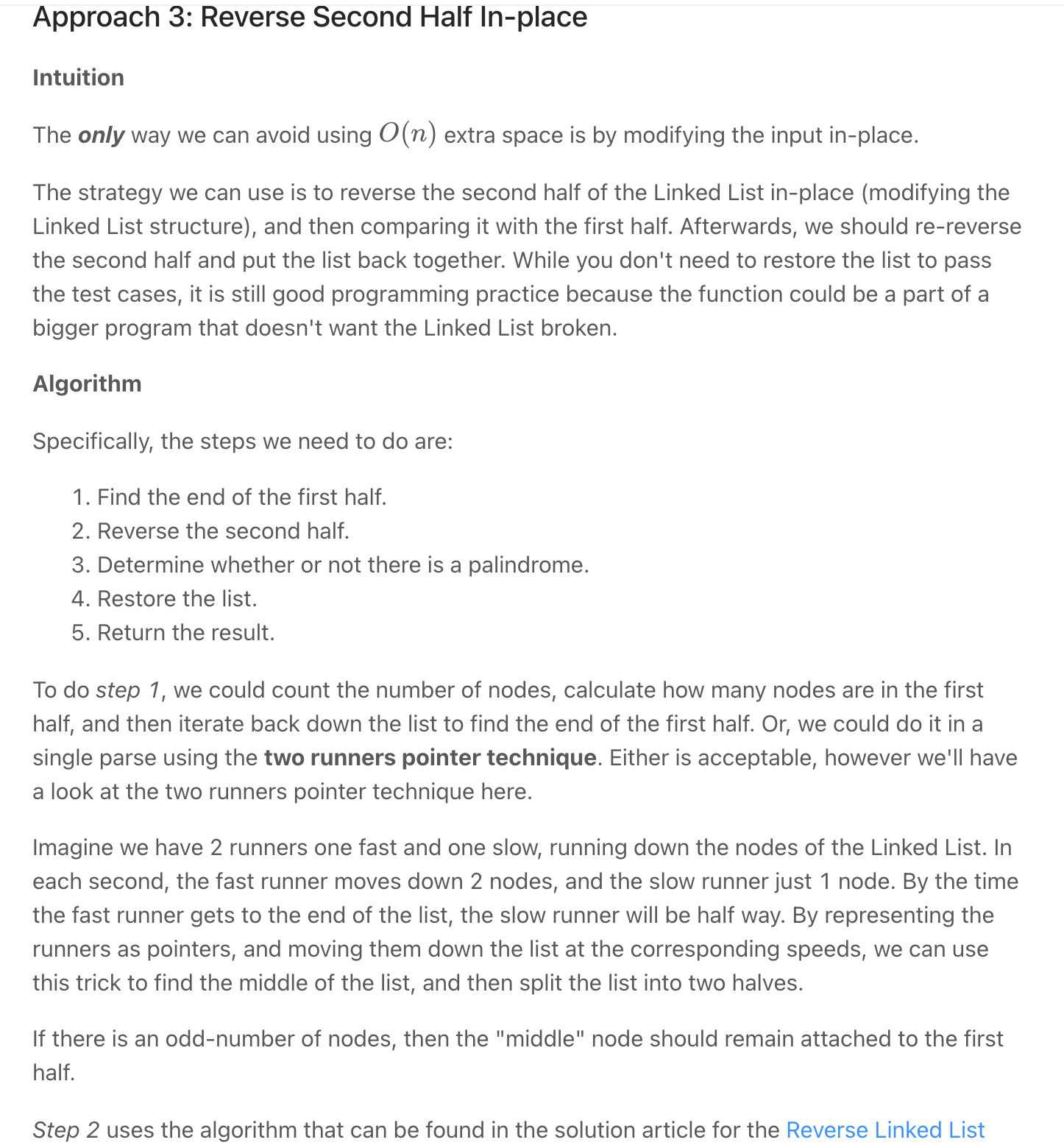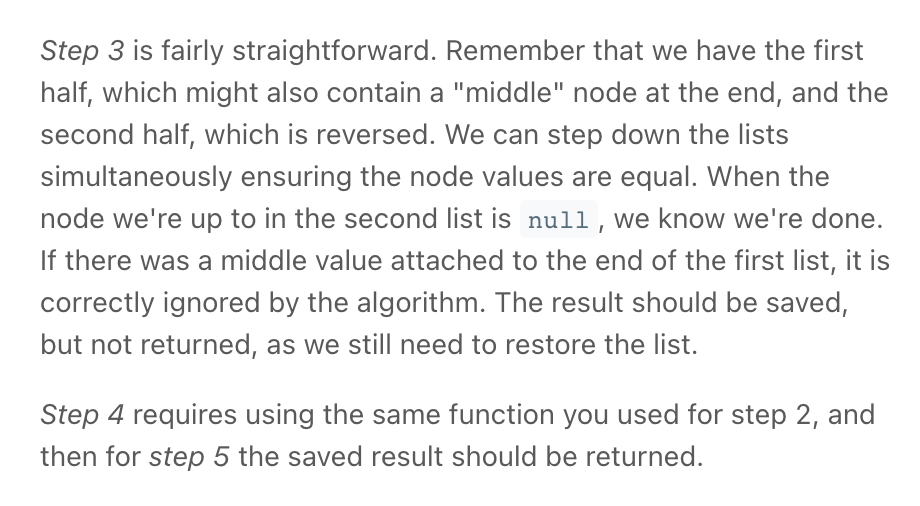• Copy List with Random Pointer *

"""
Copy List with Random Pointer:

A linked list is given such that each node contains an additional random pointer which could point to any node in the list or null.
Return a deep copy of the list.
The Linked List is represented in the input/output as a list of n nodes. Each node is represented as a pair of [val, random_index] where:
val: an integer representing Node.val
random_index: the index of the node (range from 0 to n-1) where random pointer points to, or null if it does not point to any node.

https://leetcode.com/problems/copy-list-with-random-pointer/
"""

# Definition for a Node.
class Node:
def __init__(self, x: int, next: 'Node' = None, random: 'Node' = None):
self.val = int(x)
self.next = next
self.random = random

class Solution:

# create new nodes
while node:
node.random = Node(node.val, None, node.random)
node = node.next

# populate random field of the new node
while node:
new_node = node.random
new_node.random = new_node.random.random if new_node.random else None
node = node.next

# restore original list and build new list
while node:
node.random.next = node.next.random if node.next else None
node.random = node.random.next
node = node.next

"""
"""

class Solution_:

result = Node(-1)

curr = result
store = {}
# create node
else:

# create random
new_node.random = new_random
else:

# next
curr.next = new_node
curr = new_node

return result.next

class Solution00:
def getOrCreateNodeCopy(self, store, node):
# we store the original node as a key and,
#   the new node (copy) as its value
if node not in store:
store[node] = Node(node.val)

return store[node]

res = Node(-1)
store = {}
copy = res
while old is not None:
# create old & old.random copies
node = self.getOrCreateNodeCopy(store, old)
if old.random is not None:
node.random = self.getOrCreateNodeCopy(store, old.random)

copy.next = node
copy = node

old = old.next

return res.next

• Flatten a Multilevel Doubly Linked List - LeetCode

## Tips

if it involves returning a new LL it might be easier to have a temp head (hd) then at the end return hd.next

Have a pseudo head and pseudo tail in a Doubly Linked List, so that we don't need to check the null node during the add and remove.